## Introduction

You have learnt in your earlier classes how whole numbers can be arranged in the form of different geometrical shapes.

Numbers that can be represented in the form of a square are called square numbers. E.g., 4, 9, 16 etc. Consider the square number 16. It is represented as follows:Here, you can see that there are 4 dots arranged in 4 columns. Each side of the square has 4 dots. In other words,

16 = 4 × 4 = 42

16 is called the square of 4 and 4 is the square root of 16, i.e., 4 = √16.

There are many interesting properties and patterns in square numbers. Also, there are many different applications of square roots. Watch this video to understand why squares and square roots are important.

Now you know why we should learn square and square roots.

#### Concepts

The chapter ‘Square and Square Roots’ covers the following concepts:

#### Square Numbers

You know that:In other words, a square of a number is obtained by multiplying a number with itself.

Consider the number 81. When you multiply 9 by 9, the product is 81. Hence, 81 is the square of 9.

Similarly,

1 × 1 = 1

2 × 2 = 4

3 × 3 = 9

4 × 4 = 16 and so on.

Hence, the numbers 1, 4, 9, 16…. are called square numbers. These numbers are also called as perfect square numbers.

When a natural number is multiplied by itself, the product is called a square number.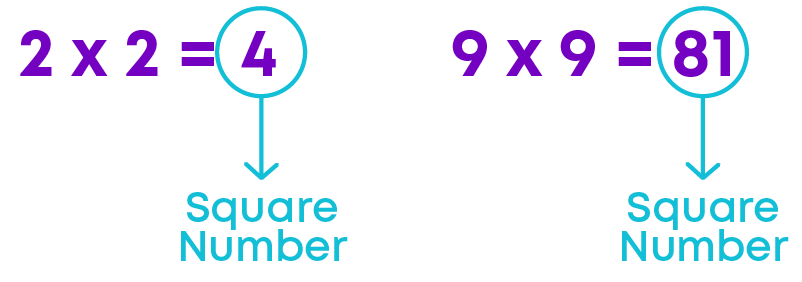We know what square numbers are. Let us learn why the numbers 1, 4, 9… are called square numbers.

Squares have all the sides equal; in a similar manner, a square number is the product of two numbers which are equal (i.e. the number itself).

Area of a square = Side × Side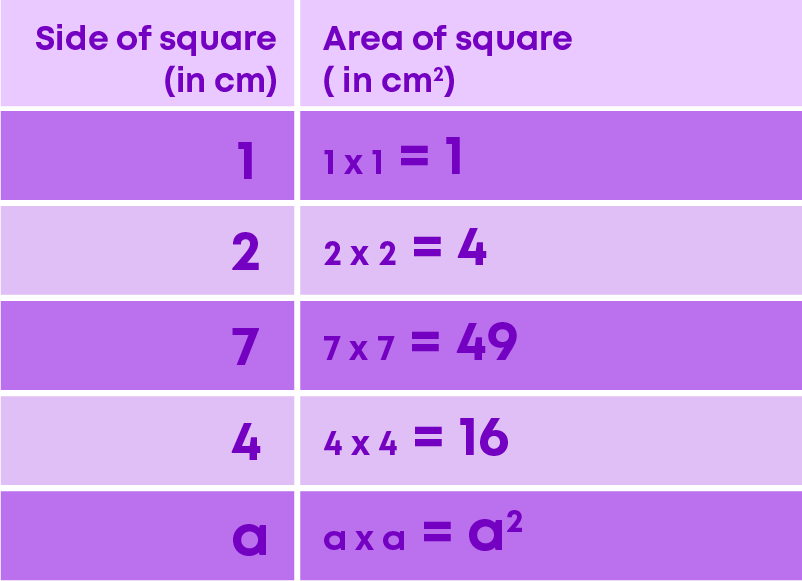If ‘a’ is a number, then its square = a × a = a2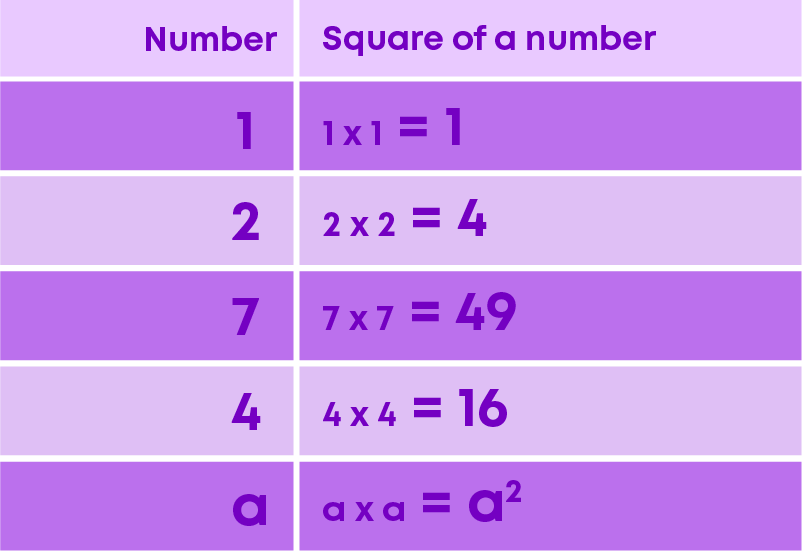In other words, the name ‘square number’ comes from the fact that these numbers of objects can be arranged to fill a perfect square, as given below.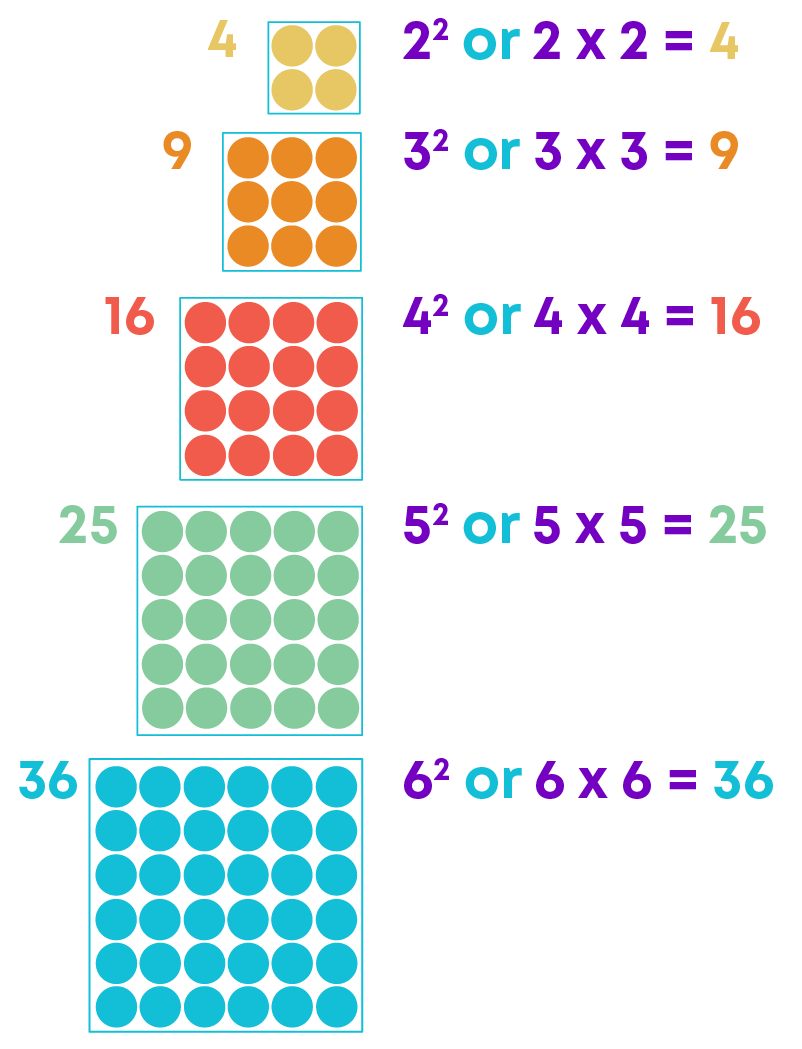Here, you see that the numbers 4, 9, 16, 25 and 36 can be arranged in the form of a square. So, these numbers are called square numbers. The square numbers from 1 to 100 are: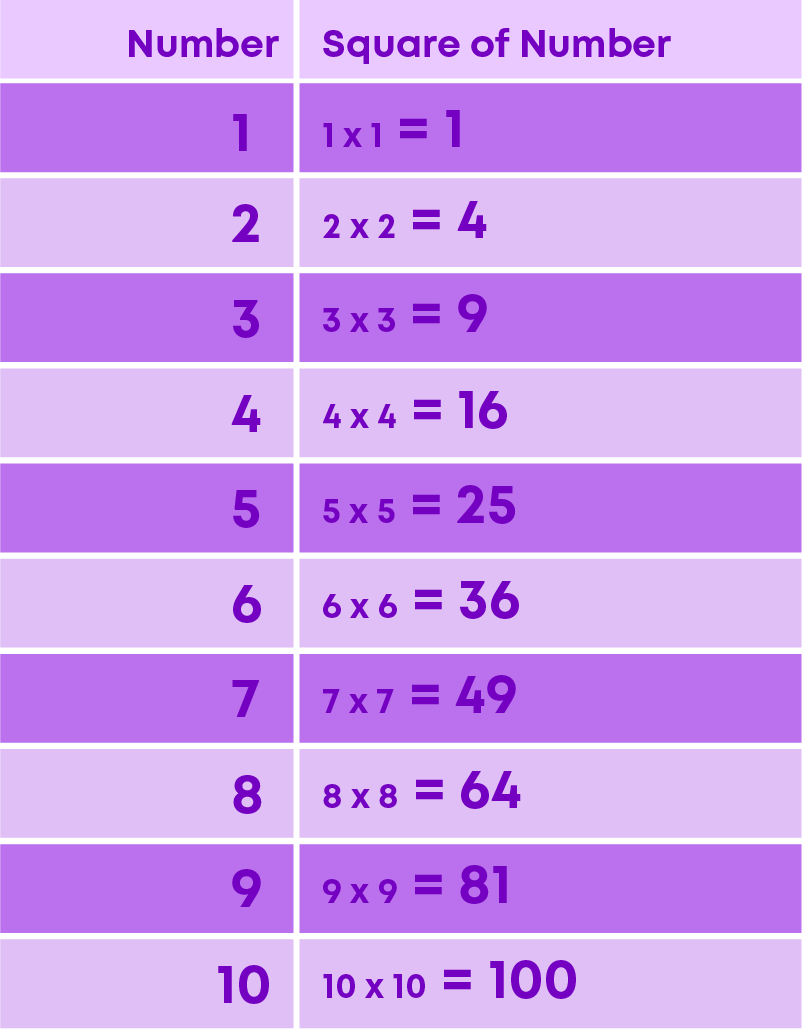From the table above, you can see that

• Square of a 1-digit number is a 1-digit number or a 2-digit number.
• Square of a 2-digit number is a 3-digit number or a 4-digit number

We know that the square of a number is obtained when we multiply a number with itself. Let us learn how to identify the square numbers based on the digit in the ones place.

Now, let us place the squares of numbers 1 to 20 in the following table: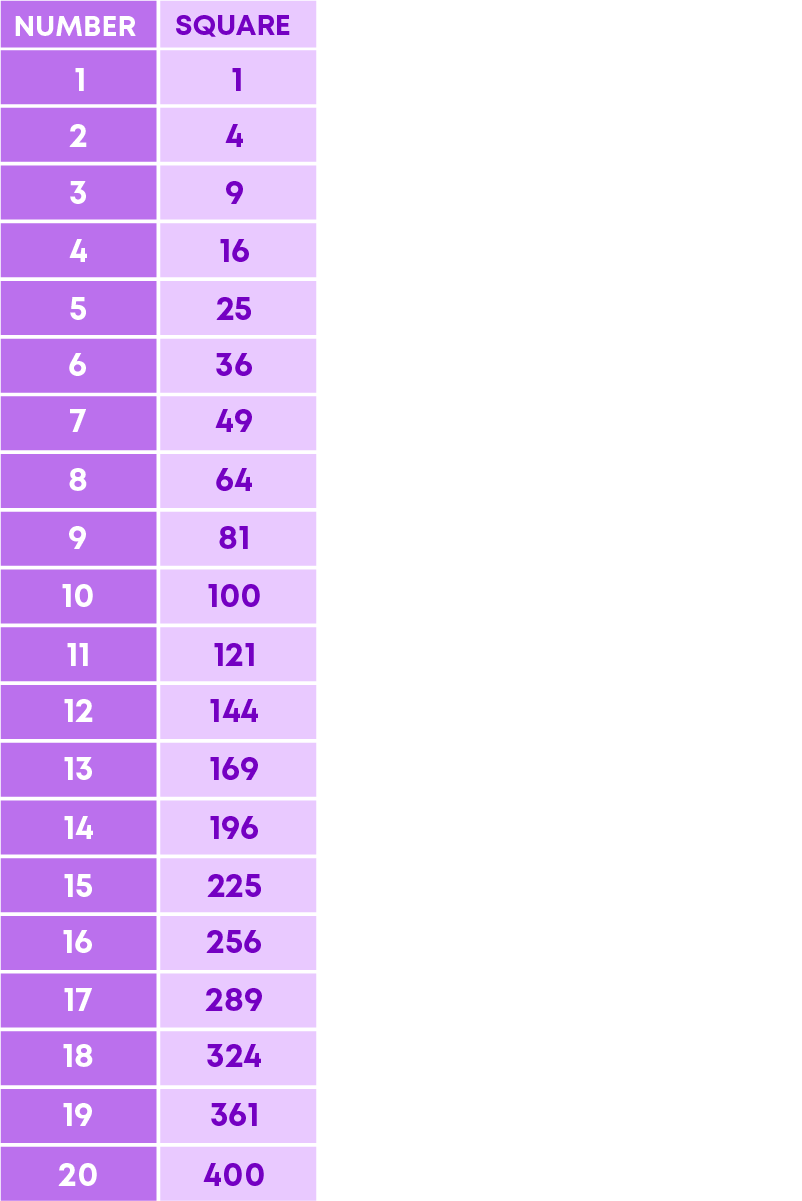What are the digits in the ones place? By observing the table, we can see that all the square numbers end with 1, 4, 5, 6, 9 or 0. They have the digits 1, 4, 5, 6, 9 or 0 at the units place. Also, 1, 4, 9, 16…., 169, 196…., 400 are perfect square numbers.

By looking at the unit’s digit of a number, we can identify whether it can be a perfect square or not. Numbers ending with 2, 3, 7, or 8 are not perfect square numbers. For example, the numbers 72, 83, 187 and 248 end in 2, 3, 7 and 8, respectively. Hence, none of them is a perfect square number.

• Let us explore square numbers which end with 1.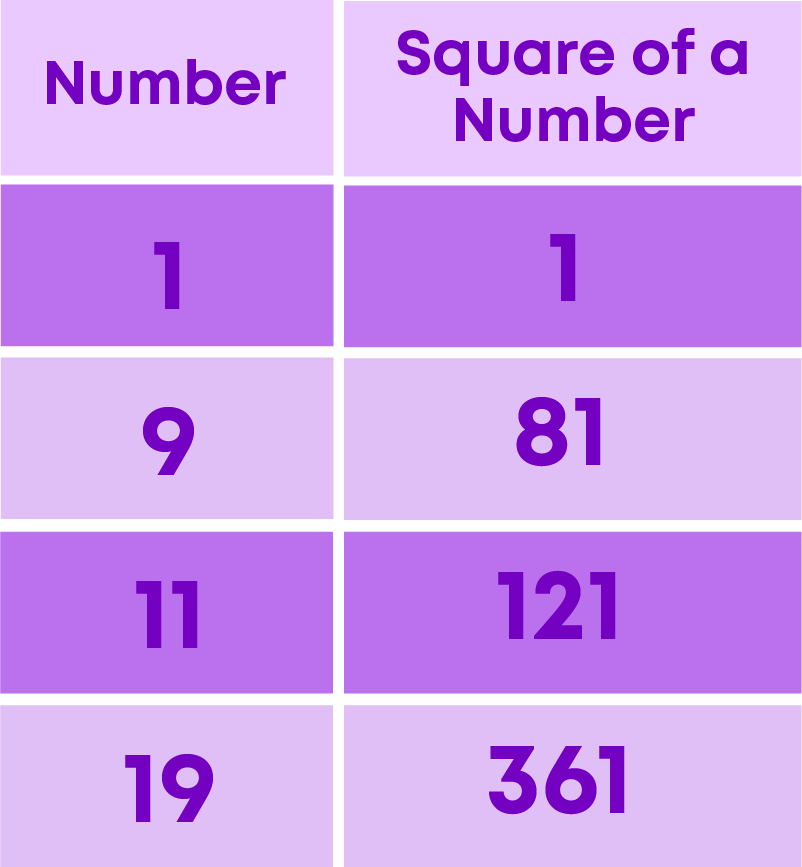If a number has 1 or 9 in the unit’s place, then its square ends with 1.

• Let us explore square numbers which end with 6.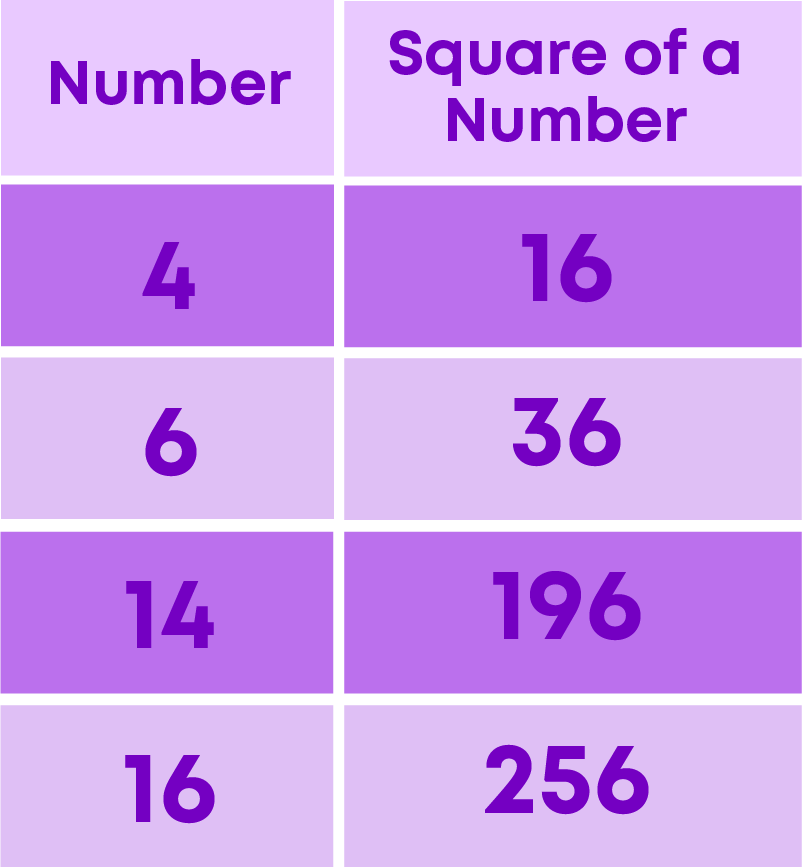If a number has 4 or 6 in the unit’s place, then its square ends with 6.

• Let us explore square numbers which end with 4.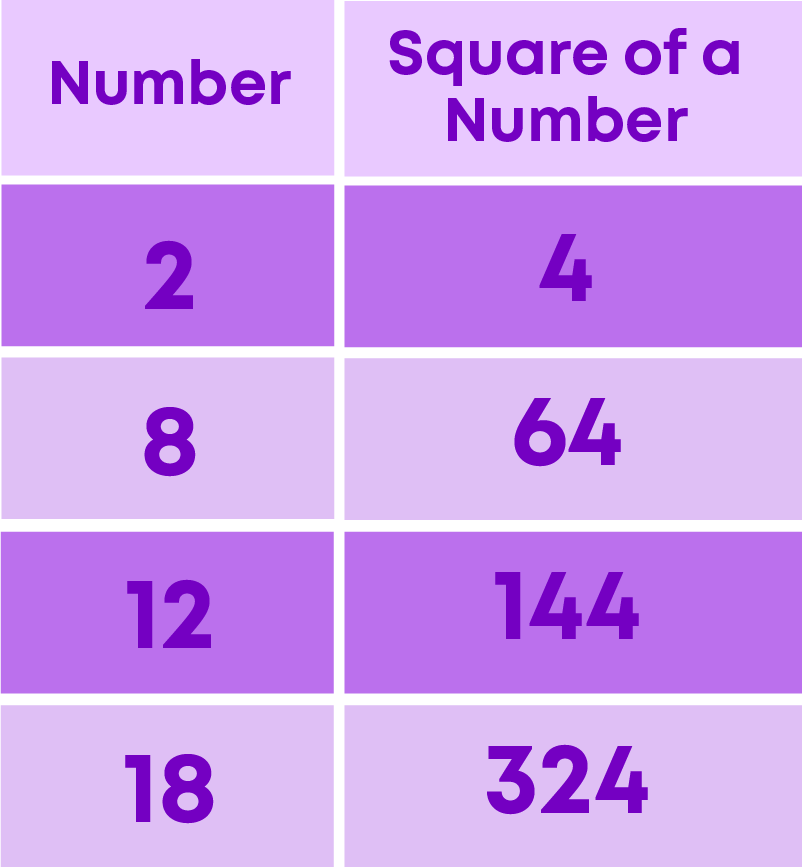If a number has 2 or 8 in the unit’s place, then its square ends with 4.

• Let us explore square numbers which end with 9.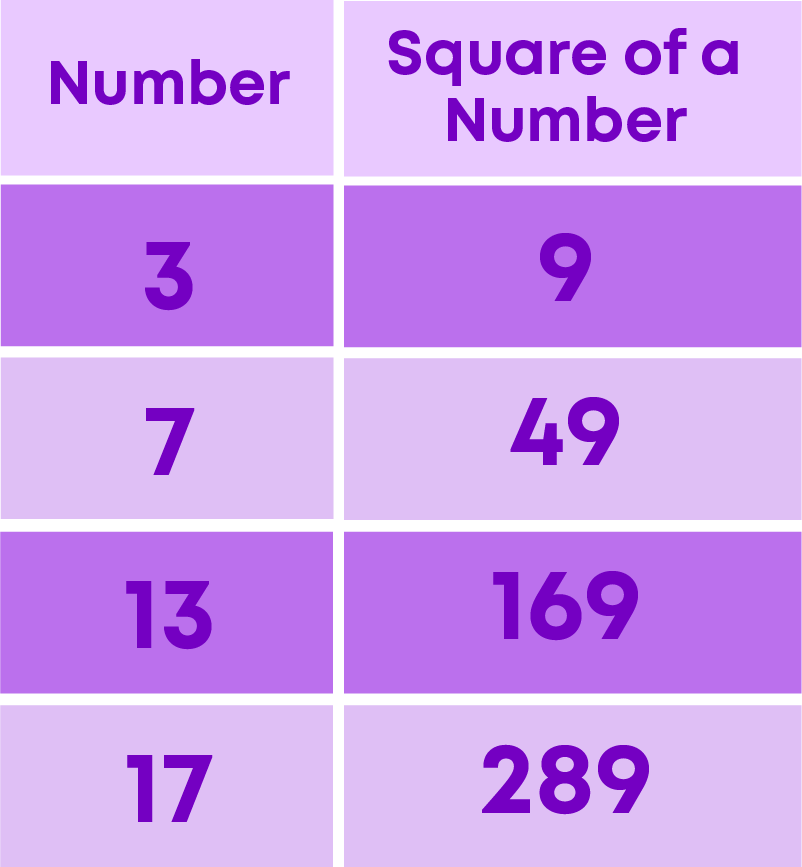If a number has 3 or 7 in the unit’s place, then its square ends with 9.

• Let us place some square numbers which end with 5.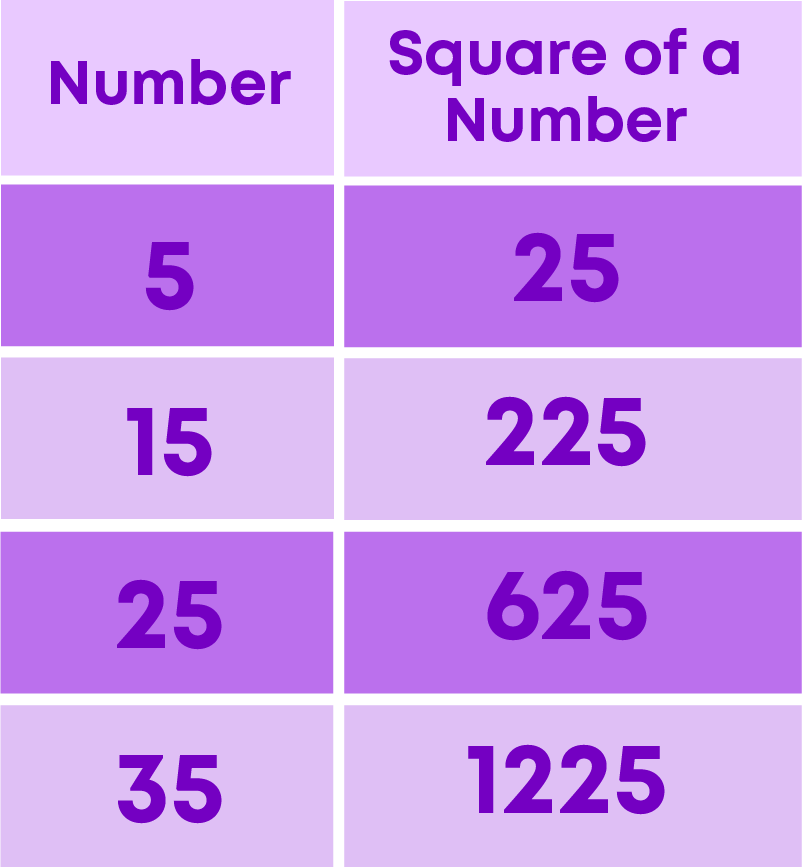If a number has 5 in the unit’s place, then its square also ends with 5.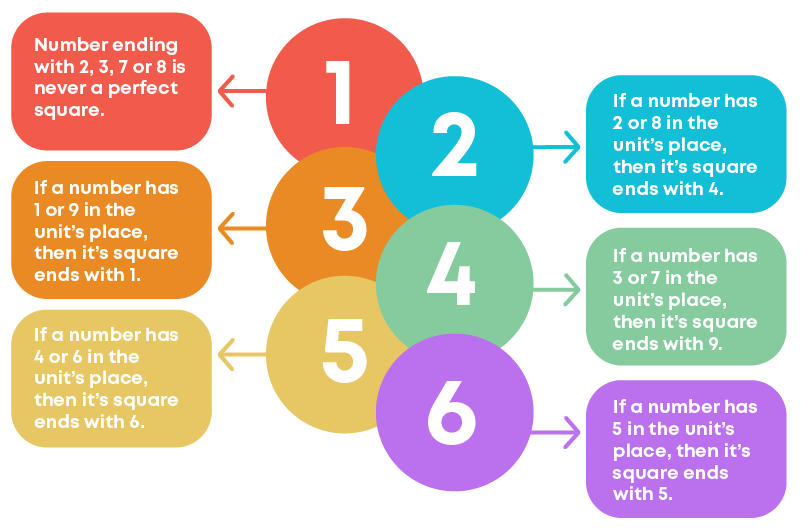As we know, the square of a number is obtained when we multiply a number with itself. Let us learn how to identify square numbers based on the ending zeroes. Below are square numbers which end with zeroes.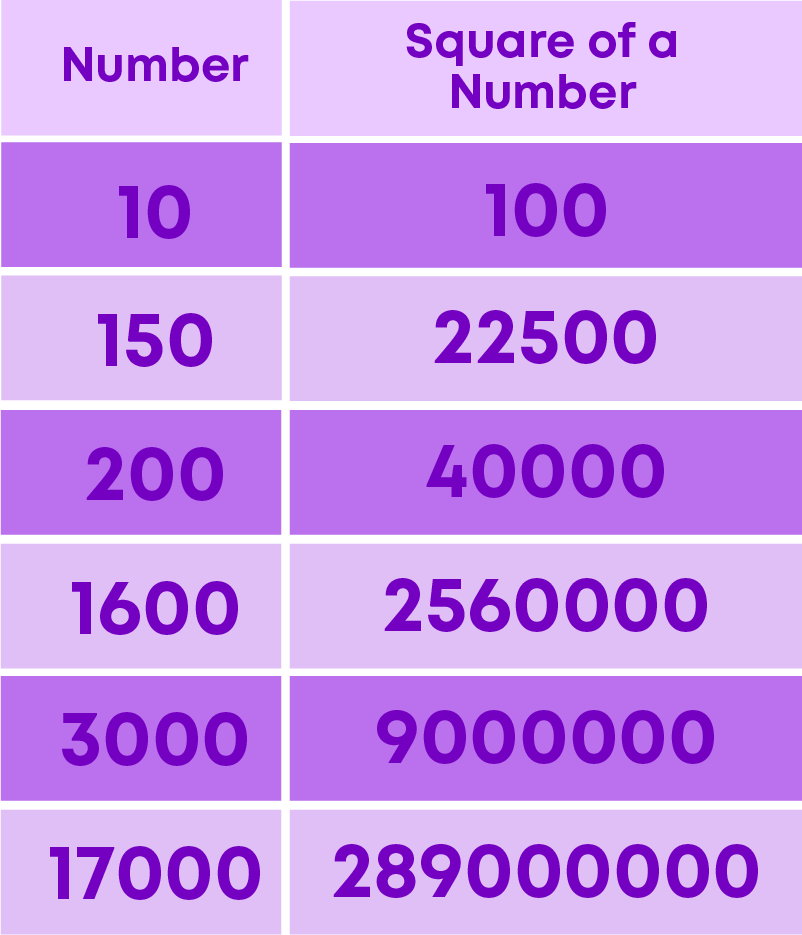Remember:

• If a number ends with one zero, then its square ends with two zeroes.
• If a number ends with two zeroes, then its square ends with four zeroes.
• If a number ends with three zeroes, then its square ends with six zeroes.

i.e., perfect square numbers always end with even number of zeroes. But all numbers ending with even number of zeroes are not perfect square numbers. E.g., 500 ends with even number of zeroes but it is not a perfect square number. Also, none of the square numbers end with one zero, three zeroes, five zeroes and so on.

Numbers ending with an odd number of zeroes are never perfect squares. For example, the numbers 10, 360, 4000 and 10000 ends with an odd number of zeroes. Hence, none of them is a perfect square.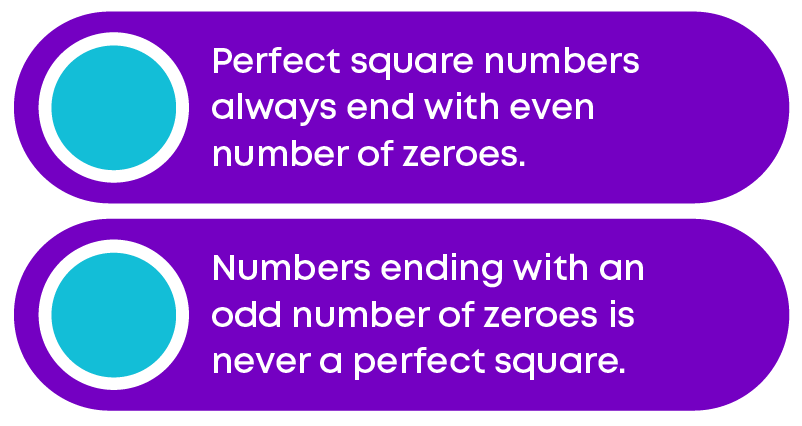You know how to find the square of a number by multiplication, and it is quite easy to find the squares of small numbers. But can we find the square of 32 so easily? To find the square of 32 without actual multiplication:

• Split the number into a sum of two numbers
• Solve using the distributive law

For example, we can split 32 as the sum of 30 and 2, i.e., 32 = 30 + 2.

322 = (30 + 2)2

= (30 + 2) × (30 + 2)

= 30(30 + 2) + 2(30 + 2)

= 900 + 60 + 60 + 4

= 1024

The square of 32 = 322 = 1024

Let us explore the patterns in squares of numbers which end with the digit 5. Consider some square numbers which end with 5.Here you can see that all squares end with 25.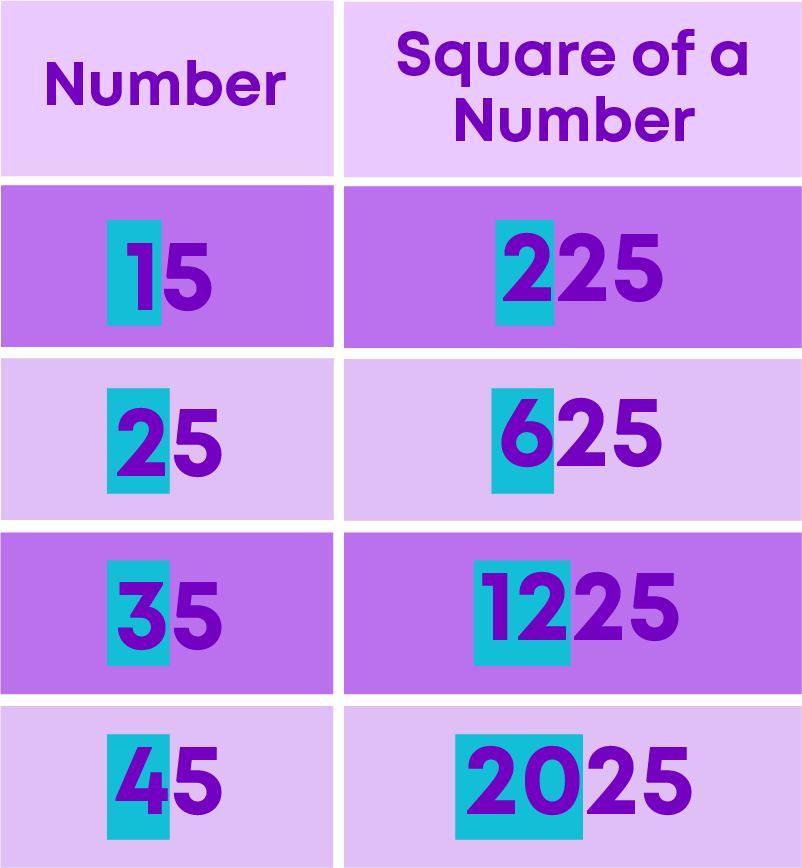Did you notice any pattern in the highlighted numbers above? Here all the squares begin with different numbers and end with 25.

Let us take 152. Check if there is any pattern.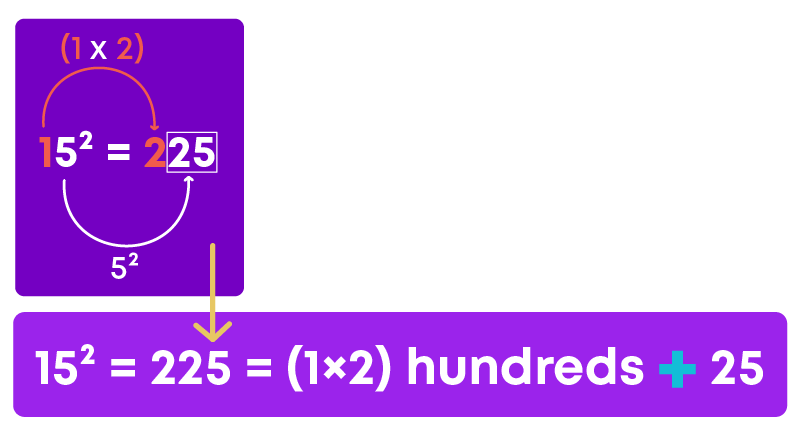Here, the digit at tens place is 1 and its successor is 2. Similarly, let us take 252.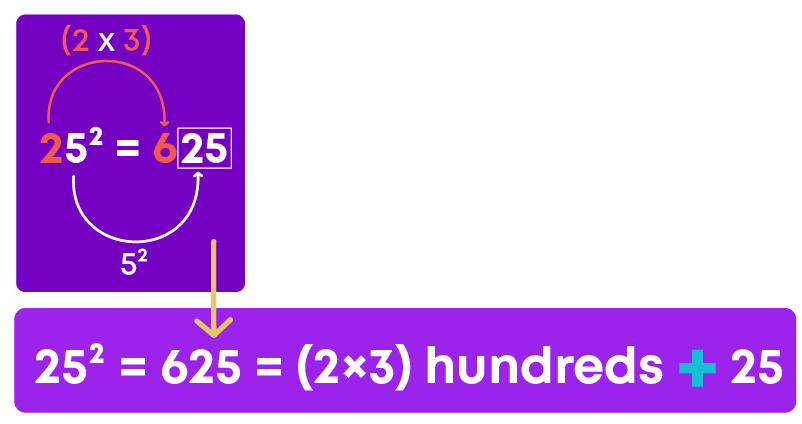Here, the digit at tens place is 2 and its successor is 3. Now, let us take 352.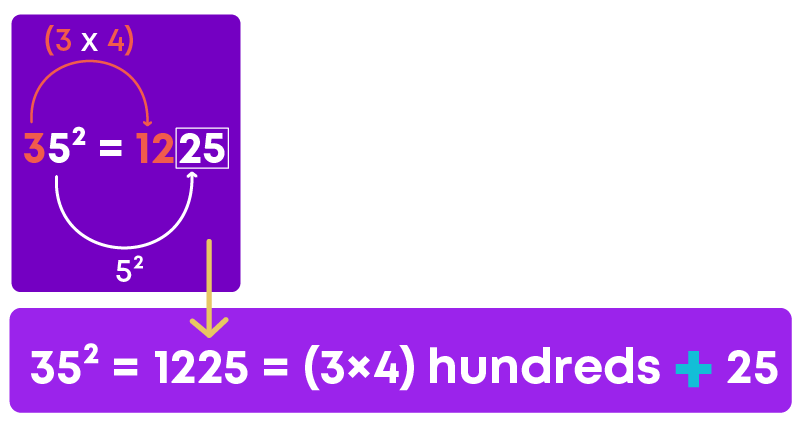Here, the digit at tens place is 3 and its successor is 4.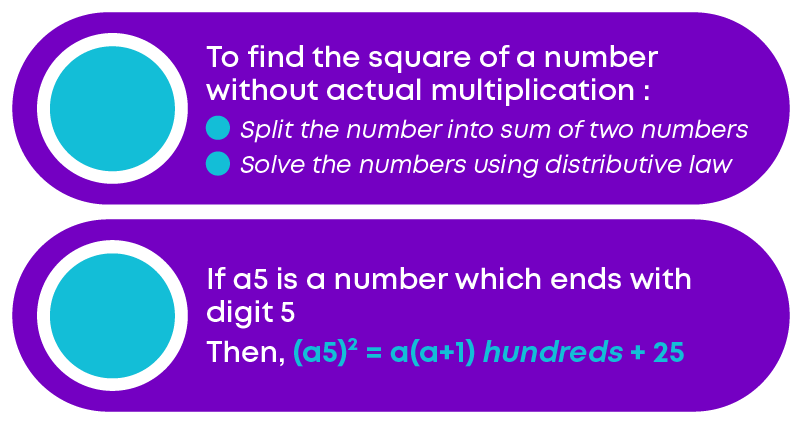A Pythagorean triplet is a set of three positive integers namely a, b, and c that represent the sides of a right triangle such that the equation a2 + b2 = c2 holds true. This is based on the Pythagorean theorem.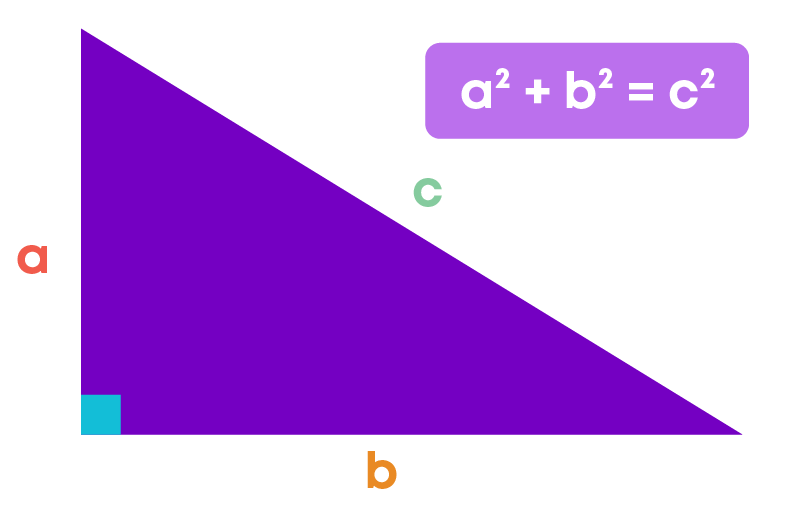There are infinite number of Pythagorean triplets. We can find them by using the Euclid formula. Let us understand how to find them.

For every natural number m > 1,

(2m)2 + (m2 – 1)2 = (m2 + 1)2

Consider m = 2

2m = 4

m2 – 1 = 22 – 1 = 3

m2 + 1 = 22 + 1 = 5

Also, we know that 3, 4 and 5 are Pythagorean triplets. Since 32 + 42 = 9 + 16 = 25,

32 + 42 = 52

Consider m = 4

2m = 8

m2 – 1 = 42 – 1 = 15

m2 + 1 = 42 + 1 = 17

Also, we know that 15, 17 and 18 are Pythagorean triplets.

82 + 152 = 64 + 225 = 289 = 172

Hence, we can conclude that for every natural number m > 1,

2m, m2 – 1, m2 + 1 are Pythagorean triplets.

• Pythagorean triples have been known since ancient times.
• Pythagorean brotherhood considered these sacred and kept it as a secret.
• Only people who were highly intellectual were given access to these numbers
• Pythagoras believed that these numbers were endowed with mystical and spiritual qualities.

#### Patterns in Square Numbers

As you know, triangular numbers are numbers that can be represented by a pattern of dots arranged as triangles. For example,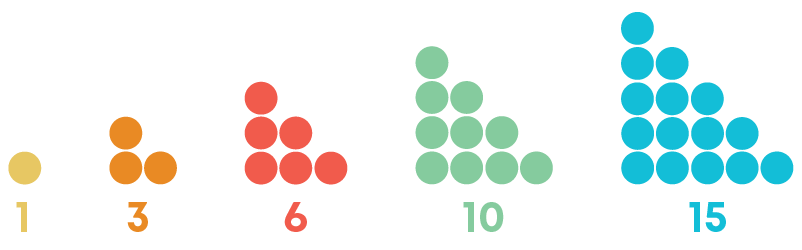Here, the numbers 1, 3, 6, 10 and 15 are triangular numbers. Now, let us combine the consecutive triangular numbers 1 and 3.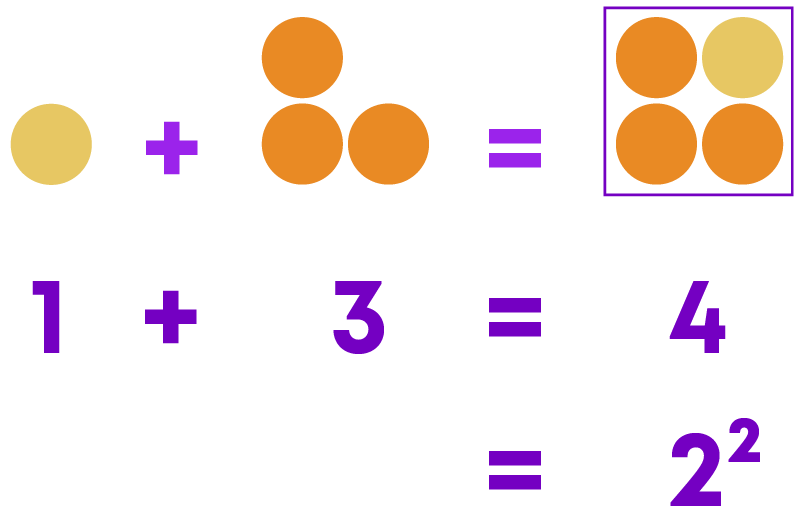Thus, we get 1 + 3 = 4 = 22

Now, let us combine the consecutive triangular numbers 3 and 6.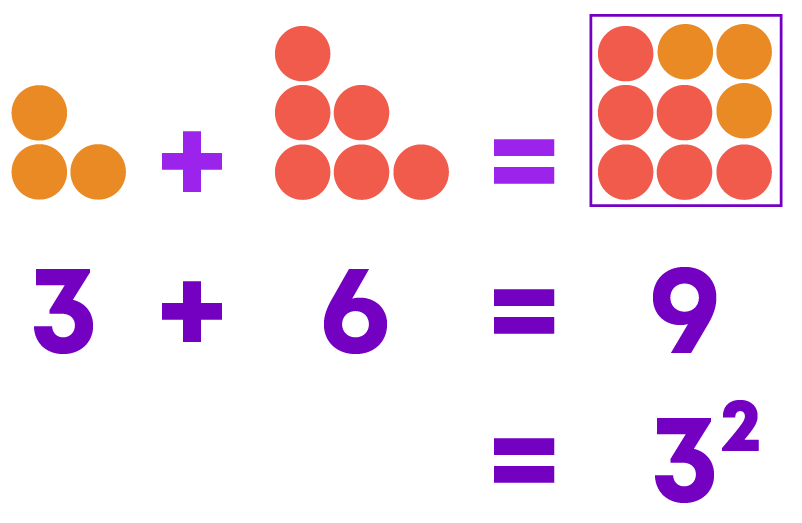Thus, we get 3 + 6 = 9 = 32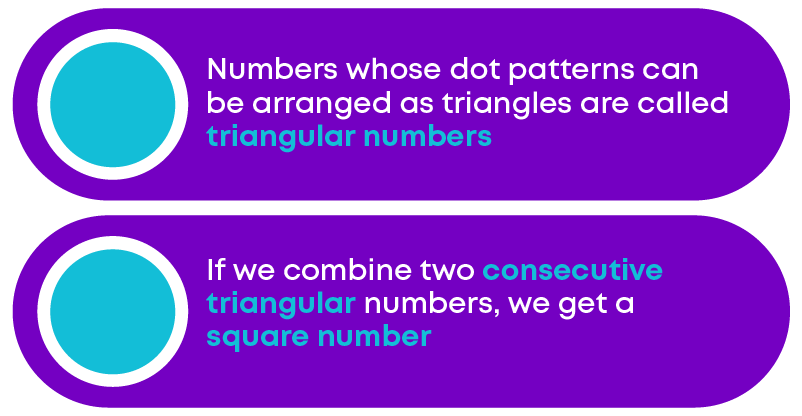So, the sum of two consecutive triangular numbers gives a square number. For example, 45 + 55 = 100 = 102

45 and 55 are consecutive triangular numbers. Their sum 100 is a square number.

Now let us express square numbers as the sum of consecutive odd numbers.

Take the first ‘n’ odd natural numbers.

• First, let us take the first odd natural number 1. 1 = 12.
• Now, let us take the first two odd natural numbers, i.e., 1 and 3. 1 + 3 = 4 = 22
• Next, let us take the first three odd natural numbers, i.e., 1, 3, and 5. 1 + 3 + 5 = 9 = 33

Hence, by observing these examples we can conclude that the sum of the first ‘n’ odd natural numbers is n2.

In other words:

If the number is a square number, then it must be the sum of the successive odd numbers starting from 1. For example, consider the number 36. Successively, subtract 1, 3, 5, 7, …. from 36.

(i) 36 - 1 = 35

(ii) 35 – 3 = 32

(iii) 32 – 5 = 27

(iv) 27 – 7 = 20

(v) 20 – 9 = 11

(vi) 11 – 11 = 0

This means, 36 = 1 + 3 + 5 + 7 + 9 + 11

i.e., we can express 36 as the sum of the successive odd numbers starting from 1.

i.e., 36 = 1 + 3 + 5 + 7 + 9 + 11

Let us take another number 27. Successively, subtract 1, 3, 5, 7, …. from 27.

(i) 27 - 1 = 26

(ii) 26 – 3 = 23

(iii) 23 – 5 = 18

(iv) 18 – 7 = 11

(v) 11 – 9 = 2

(vi) 2 – 11 = -9

Here, we are not able to express 27 as the sum of the successive odd numbers starting with 1. Hence, we can say that,

If a natural number cannot be expressed as the sum of successive odd natural numbers starting with 1, then it is not a perfect square.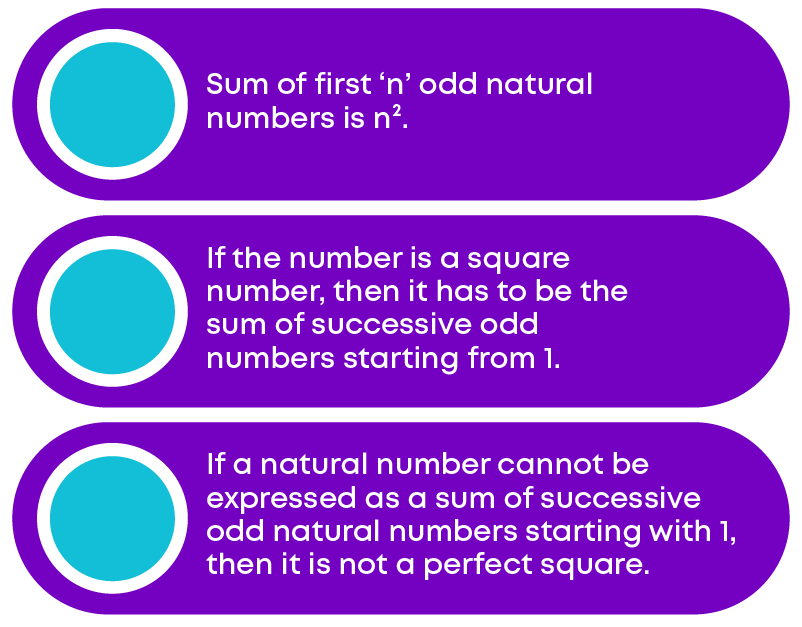Now let us express square numbers as the sum of two consecutive natural numbers. Consider an odd number 3. 32 = 9. Also, we know that 9 = 4 + 5. Hence, we can write it as,

32 = 9 = 4 + 5

Now, consider another odd number 5. 52 = 25. Also, we can express 25 = 12 + 13. Hence, we can write it as,

52 = 25 = 12 + 13

As we know, the square of odd numbers can be expressed as the sum of consecutive natural numbers. Now, let us generalise this rule for the square of any odd number. We have,

32 = 9 = 4 + 5

52 = 25 = 12 + 13

72 = 49 = 24 + 25

and so on. First, let us observe,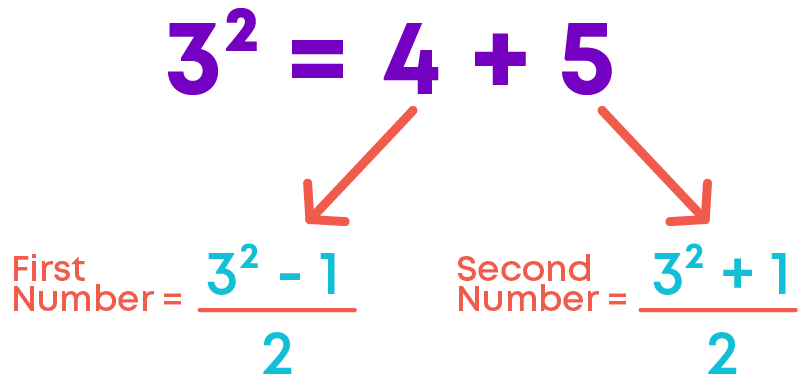Similarly,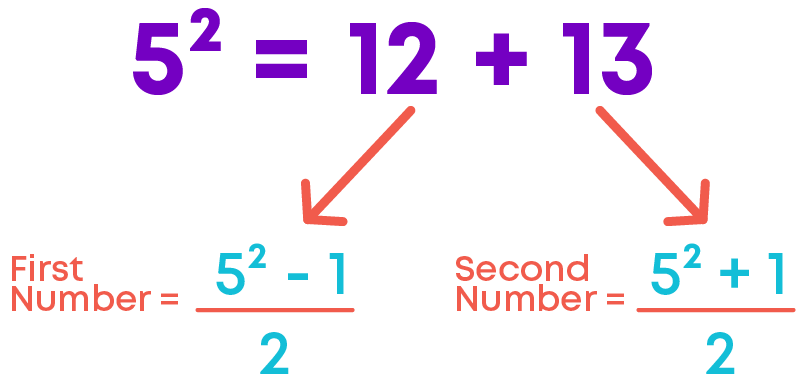If ‘n’ is any odd number, then its square can be expressed as the sum of n2- 1 2 and 2 n2+ 1 2

For example, 72 = 49 = 24 + 25 = 72- 1 2 + 72+ 1 2

We know that any odd number can be expressed as a sum of two consecutive natural numbers. What about the converse? Is the converse true? That is,

Is the sum of any two consecutive natural numbers the perfect square of a number? For example,

5 + 6 = 11, but 11 is not a perfect square.

11 + 12 = 23, but 23 is not a perfect square.

Hence, we cannot say that the sum of any two consecutive natural numbers is always a perfect square.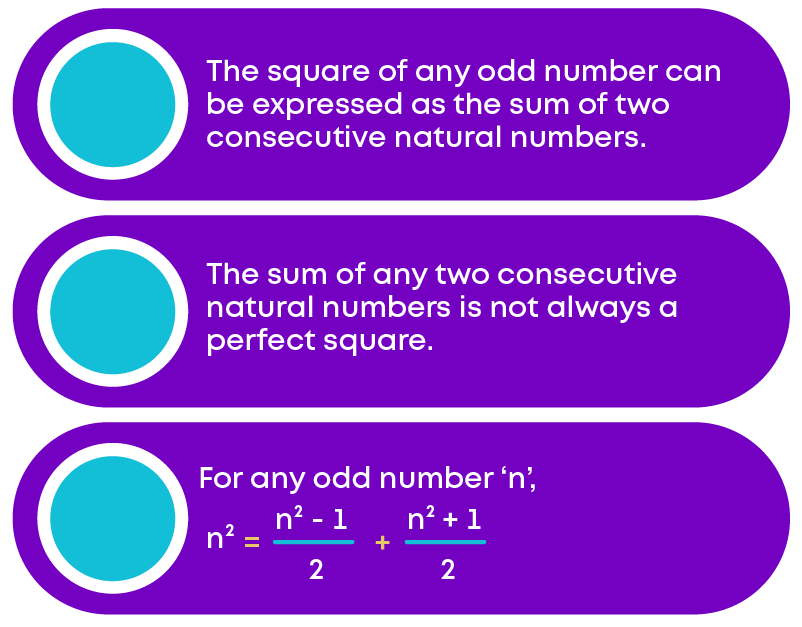Now, let us explore the product of consecutive even / odd numbers. In the previous questions, you have seen that

7 × 9 = 82 – 1

6 × 8 = 72 – 1

Do you observe any pattern here? Let us take some more consecutive even/ odd numbers. For example, take the consecutive odd numbers 13 and 15.

13 × 15 = 195 = 142 – 1

Now, let us observe this.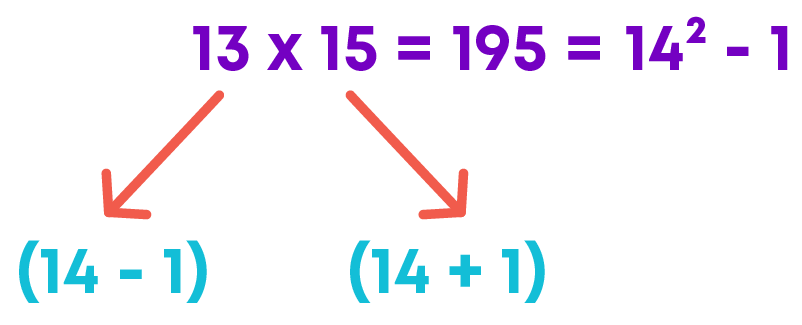So, we can write,

13 × 15 = (14 – 1)(14 + 1) = 142 – 1

Let us take two consecutive even numbers 12 and 14.

12 × 14 = 168 = 132 – 1

Now, let us observe this.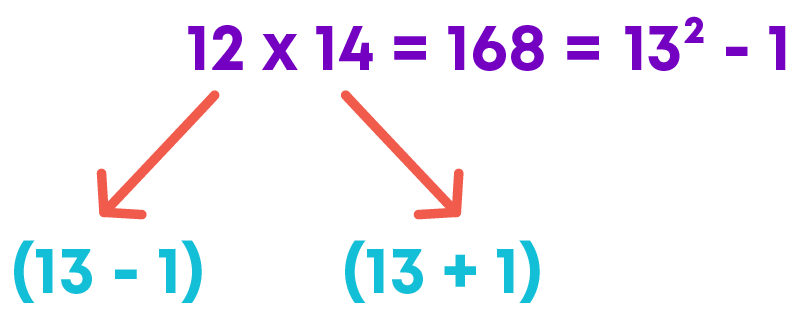So, we can write,

12 × 14 = (13 – 1)(13 + 1) = 132 – 1The pattern helps to find the product of consecutive even and odd natural numbers.

We already know interesting patterns in numbers. Now, let us explore patterns in square numbers. Observe the below pattern of square numbers.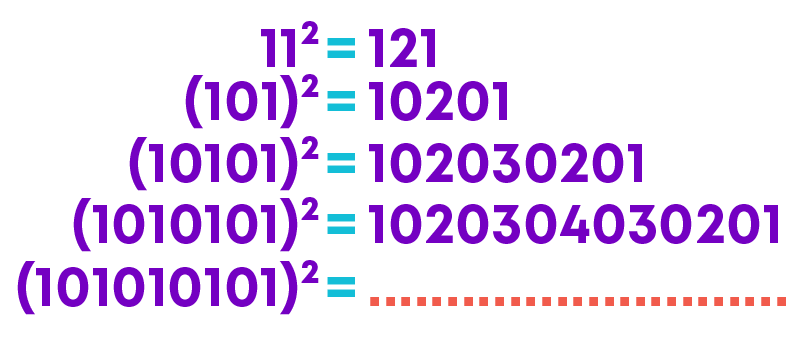What do you notice?

• 1012 = 10201

10201 has two 1s. So, in the square we write numbers from 1 to 2 and then in reverse order up to 2, putting ‘0’ in between each pair.

• (10101)2 = 102030201

10101 has three 1s. So, in the square we write numbers from 1 to 3 and then in reverse order up to 3, putting ‘0’ in between each pair. Similarly, you can complete the pattern.

Let us take another pattern.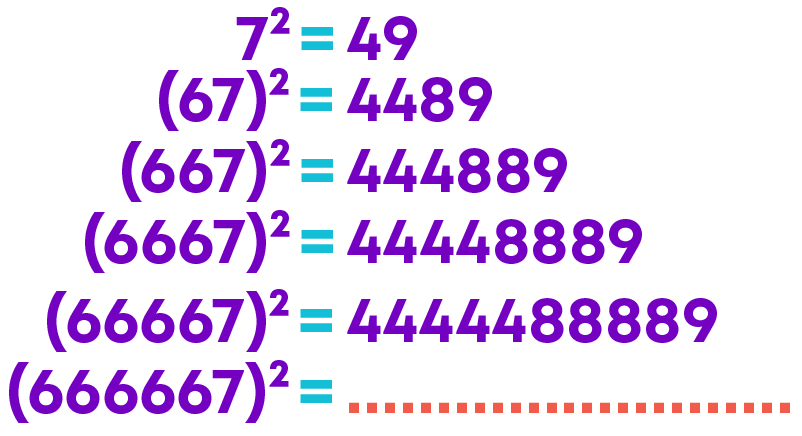What do you notice?

• 672 = 4489

67 has 1 six and 1 seven. Its square has 2 fours, 1 eight and 1 nine.

• 6672 = 444889

667 has 2 sixes and 1 seven. Its square has 3 fours, 2 eights and 1 nine.

Similarly, you can complete the pattern.

Observe these interesting patterns: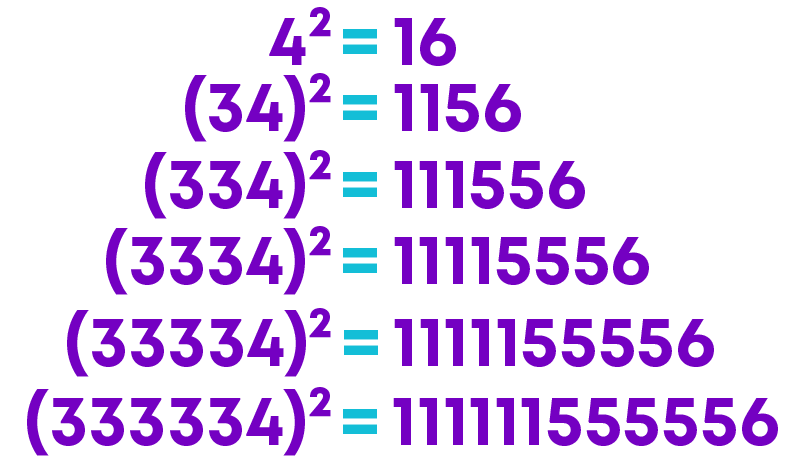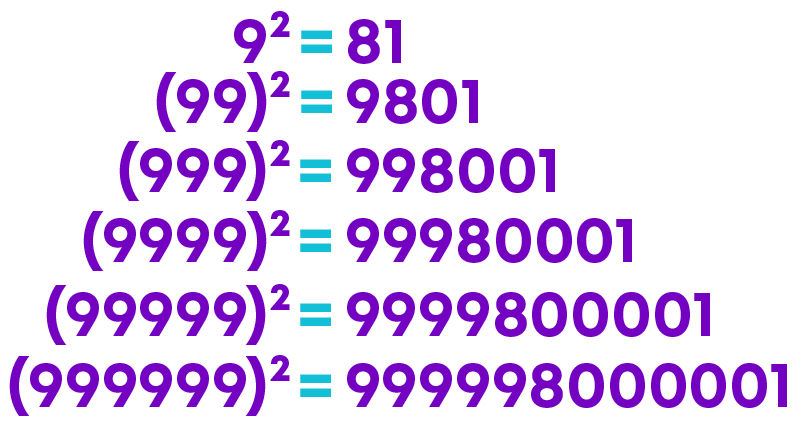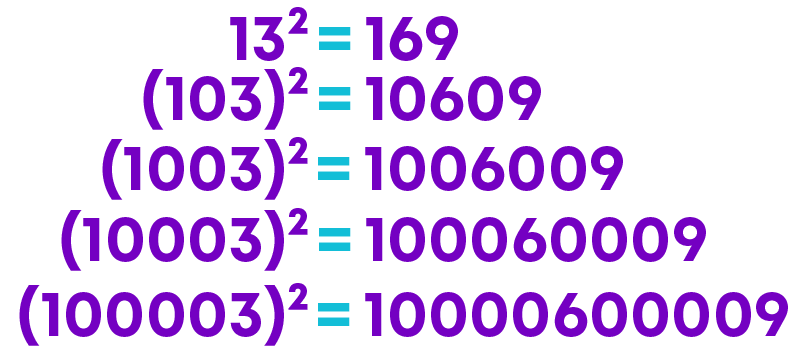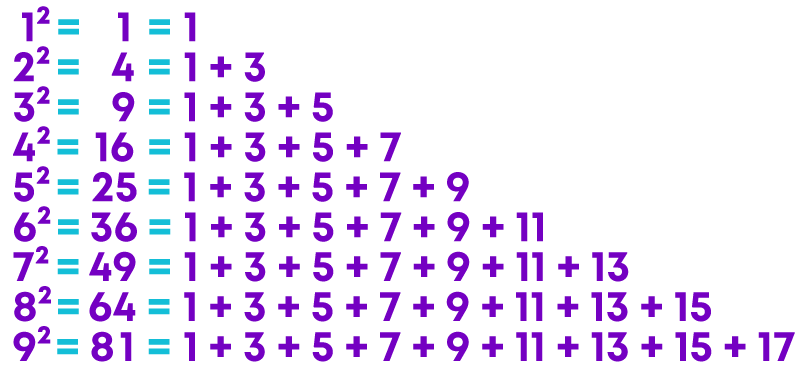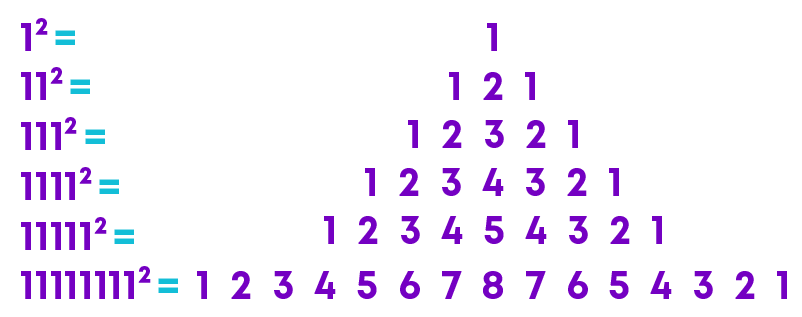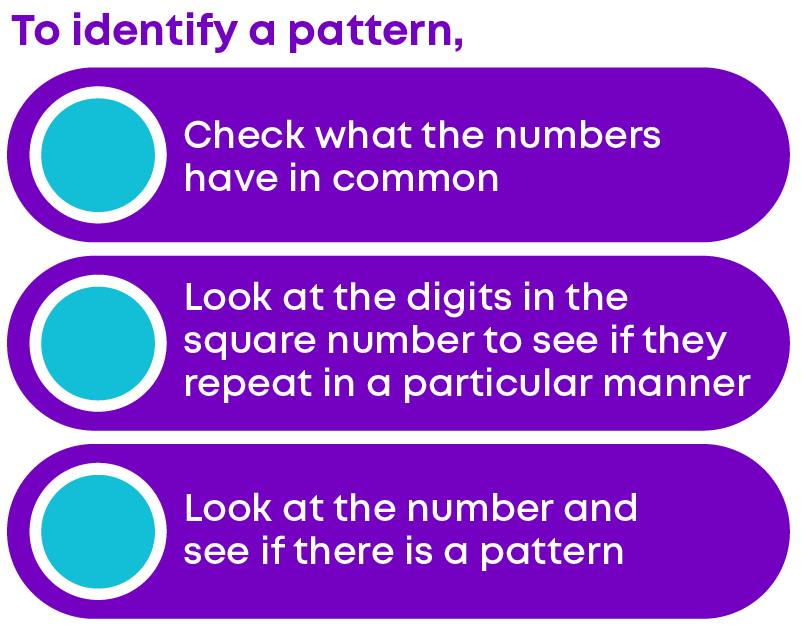We know that 1, 4, 9, 16, 25… are square numbers. Also, here 1 and 4, 4 and 9, 9 and 16 are consecutive square numbers. Let us explore an interesting pattern in these consecutive square numbers.

Let us take square numbers 1 and 4:

Now, we can check how many numbers are there between these square numbers.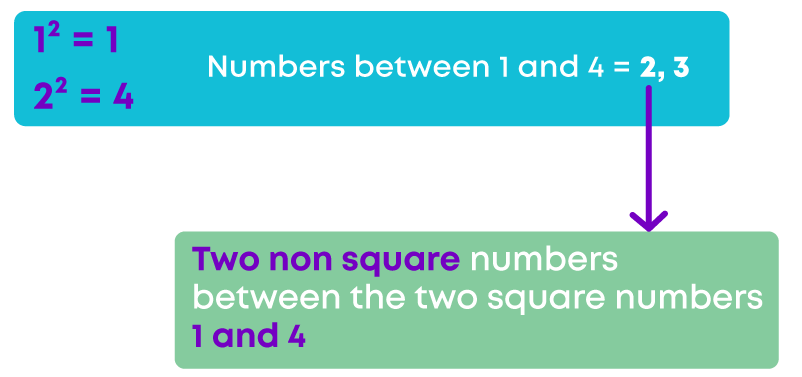There are two non-square numbers present between two square numbers 1 and 4. Also, 22 – 12 = 4 – 1 = 3

Now, let us take square numbers 4 and 9.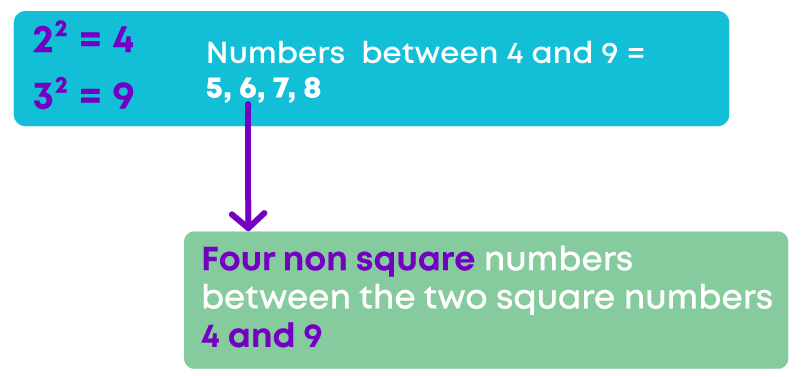Hence, there are four non square numbers present between the two square numbers 4 and 9.

Also, 32 – 22 = 9 – 4 = 5

Now, let us take the square numbers 9 and 16.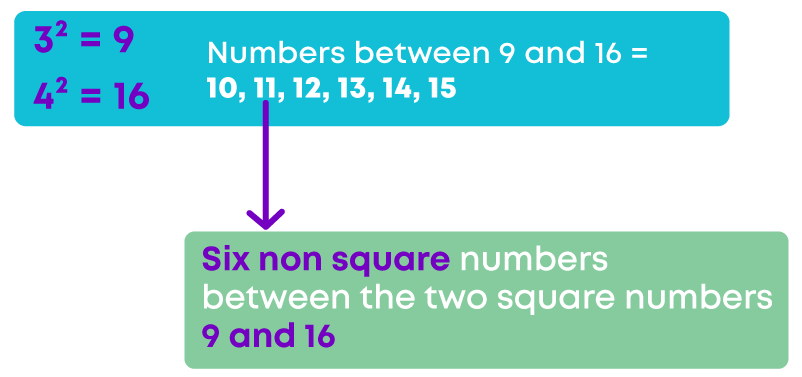Hence, there are six non-square numbers present between the two square numbers 9 and 16.

Also, 42 – 33 = 16 – 9 = 7

Remember, if ‘n’ is any natural number, then there are 2n non-square numbers between the squares of the numbers ‘n’ and (n + 1).

For example, let us take n = 5 then (n + 1) = 6 and, 2n = 10

Hence, there are 10 non square numbers present between the squares of the numbers 5 and 6.

#### The Square Root of a Number

You know that area of the square of side ‘a’ units is a × a or a2 sq. units. Consider this scenario.

Deepa knows that the area of a square is 2025 sq. cm. She wants to know the measure of the side of the square. How will she find it?So, Deepa must find the number which when multiplied by itself gives 2025. But what is that number?

Abhay knows that the diagonal of a square is 12 cm. He wants to find the side of the square. How will he find it? He used Pythagoras theorem to solve it.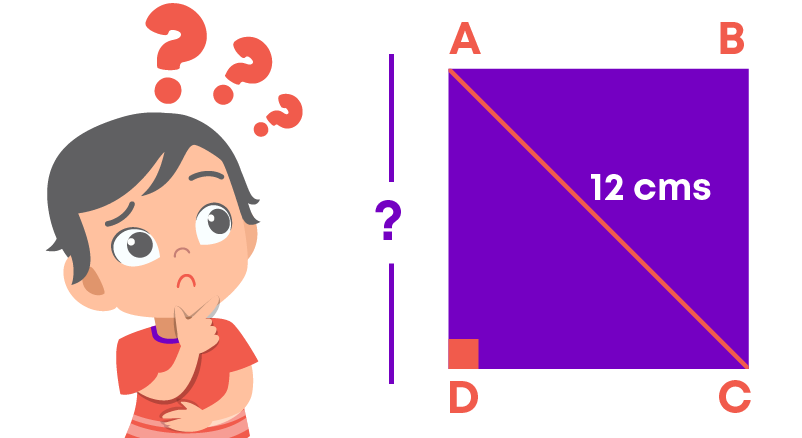Let AB = CD = x cm

x2 + x2 = 122

2x2 = 144

x2 = 72

So, Abhay needs to find the number which when multiplied by itself gives 72.

In both the cases above, the square of the number is known and Deepa and Abhay need to find the number which when multiplied by itself gives that square number.

In other words, they should find the square root of the given number.

The square root of a number is a value that, when multiplied by itself, gives the number.

You know that 9 = 3 × 3 = 32

Hence, the square root of 9 is 3. This can be denoted as √9 = 3.

You know that 32 = 9. Similarly, (-3)2 = 9. Hence, 9 has two integral roots, 3 and -3.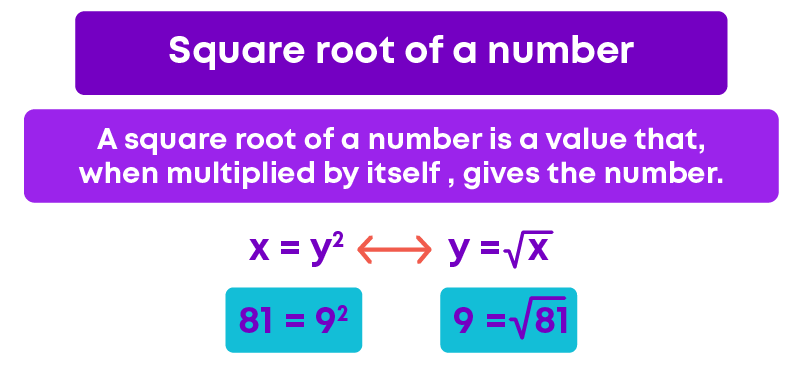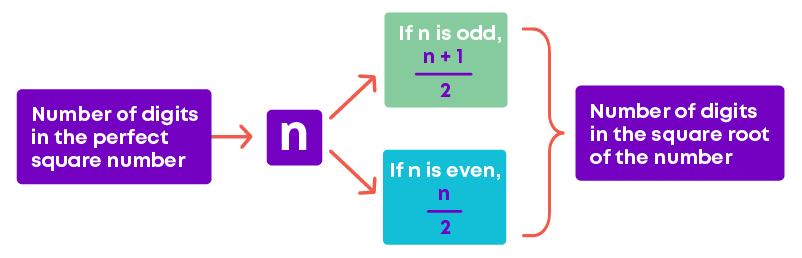For example, if a perfect square has 6 digits, then its square root will have 6 2 = 3 digits.

If a perfect square has 5 digits, then its square root will have 5+12 2 = 3 digits.

The square root of any number which is not a perfect square can be approximately calculated. This is done using the steps given below. For example, let us see how to estimate the square root of 125.

• Find two perfect squares between which the given number lies.

We know that 112 = 121 and 122 = 144

125 lies between 121 and 144.

121 < 125 < 144

Hence, 11 < √125 < 12

• Identify which perfect square is the given number closer to.

Between 121 and 144, 125 is closer to 121 than 144.

• The square root of the given number is approximately equal to the square root of the closest perfect square.

Since 125 is closer to 121, √125 is approximately equal to 11.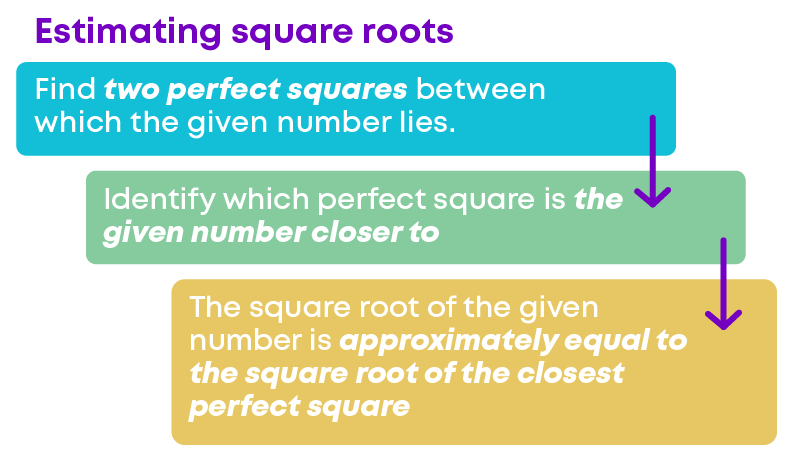You know that the sum of the first ‘n’ odd natural numbers is n2. For example, let us consider the sum of the first 6 odd numbers: 1 + 3 + 5 + 7 + 9 + 11 = 36 = 62

You know that subtraction is the opposite of addition. So, let us try to find the square root of 36 by repeated subtraction.

To find the square root of a number:

• Subtract the first odd natural number 1 from the given number.
• Subtract the second odd natural number 3 from the difference obtained in the previous step.
• Continue the process until the difference is 0.
• Count the number of times subtraction was performed.
• The count gives the square root of the given number.

Square root of 36 by repeated subtraction is as follows:

1. 36 – 1 = 35

2. 35 – 3 = 32

3. 32 – 5 = 27

4. 27 – 7 = 20

5. 20 – 9 = 11

6. 11 – 11 = 0

The repeated subtraction is done 6 times. Hence, √36 = 6.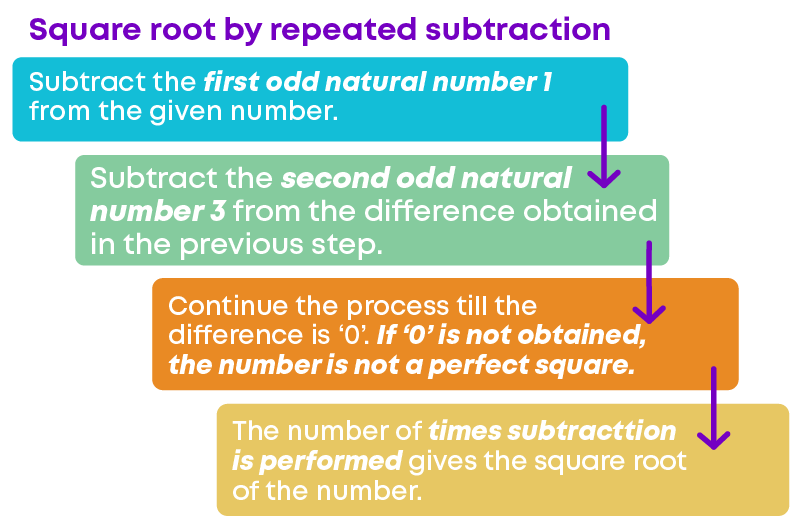Now, let us explore the prime factorisation of a given number and its square.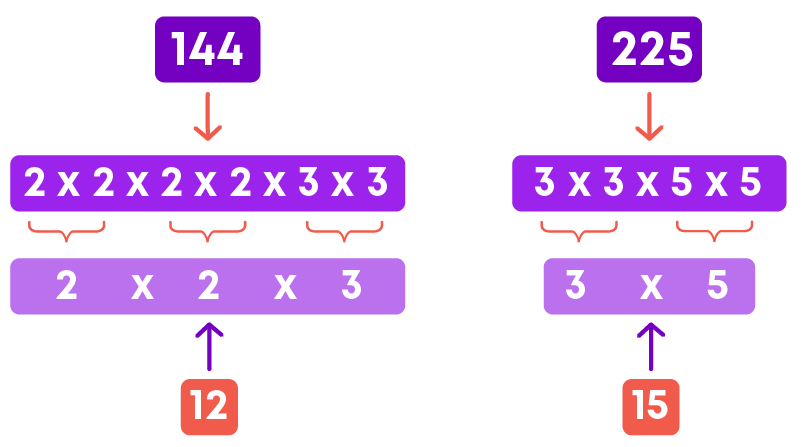How many times does a prime factor occur in the number and how many times the same prime factor occur in its square? Can you identify a pattern?

We can identify that each prime factor in the prime factorisation of the square of a number, occurs twice the number of times. It occurs in the prime factorisation of the number itself.

Let us explore the prime factorisation of some square numbers.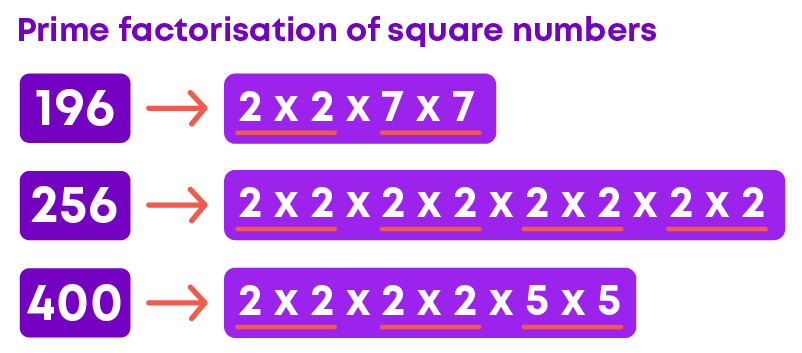We can see that in the prime factorisation of square numbers, there are pairs of prime numbers. What about numbers which are not perfect squares?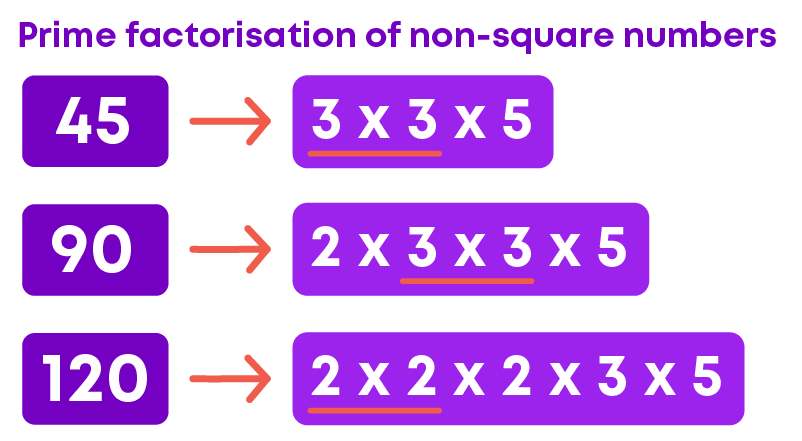Here we can see that the prime factorisation of non-square numbers has prime numbers that do not occur in pairs.

From the previous examples, we can identify if the given number is a perfect square or not by looking at its prime factorisation.

A number is a perfect square only if its prime factorisation has prime numbers that occur in pairs.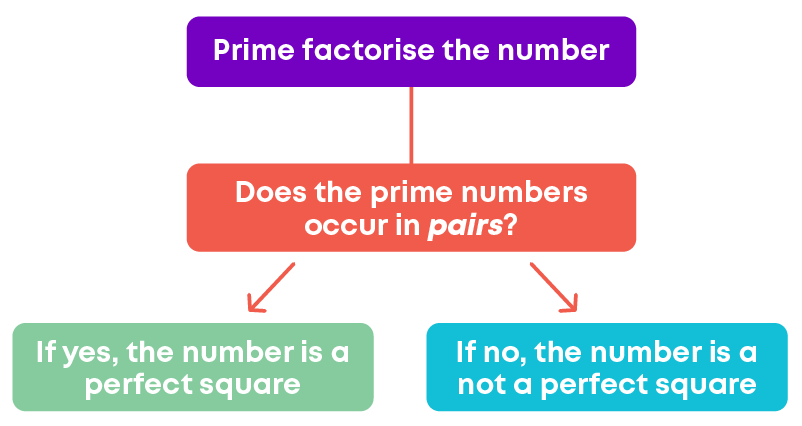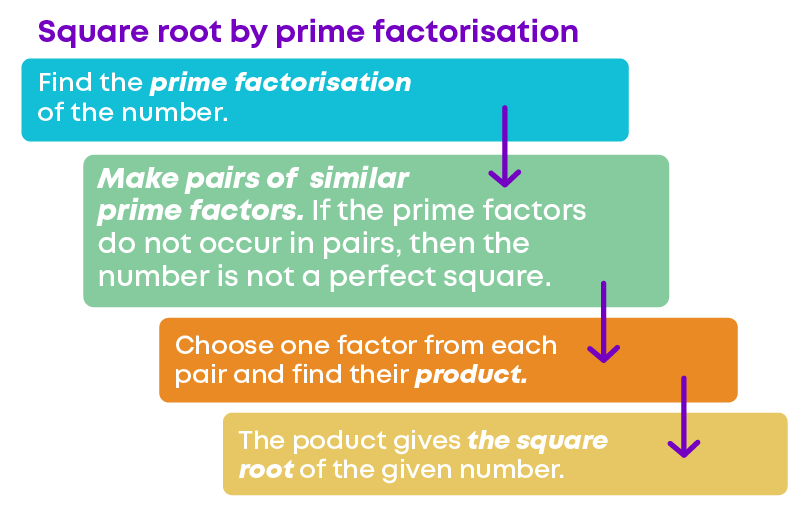You know how to find the square root of a number by the,

• Method of repeated subtraction
• Method of prime factorisation.

So, how will you find the square root of 9409? Even though you can use any of the above methods, they will be long and time-consuming. For bigger numbers, we find the square root by the long division method.

Step 1: Place a bar over every pair of digits starting from right to left.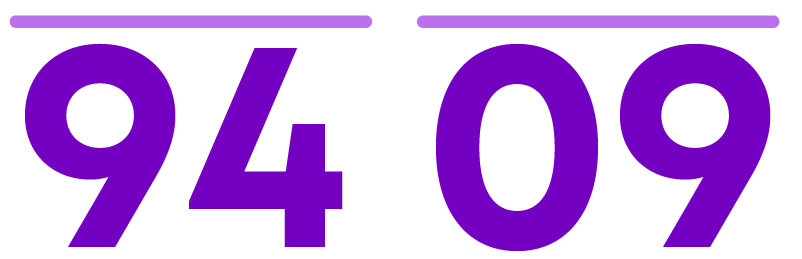Step 2: From the left consider the first pair, i.e., 94. Find the largest number whose square is less than or equal to 94.

The number is 9 (92 < 94 < 102)

Take 9 as the divisor and 94 as the dividend. Divide and get the remainder.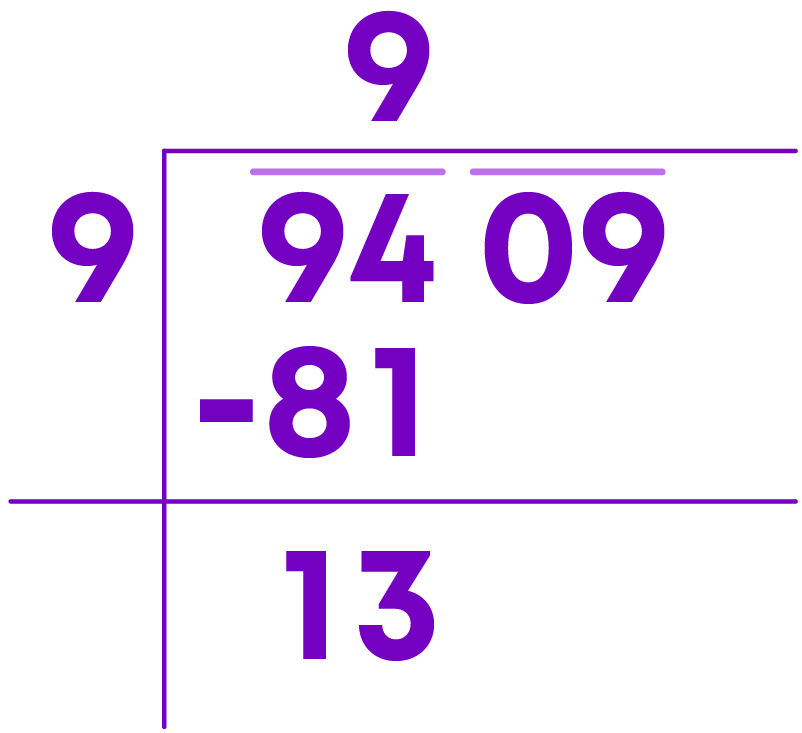Step 3: Bring down the next pair of numbers to the right of the remainder. This new number 1309 is the new dividend.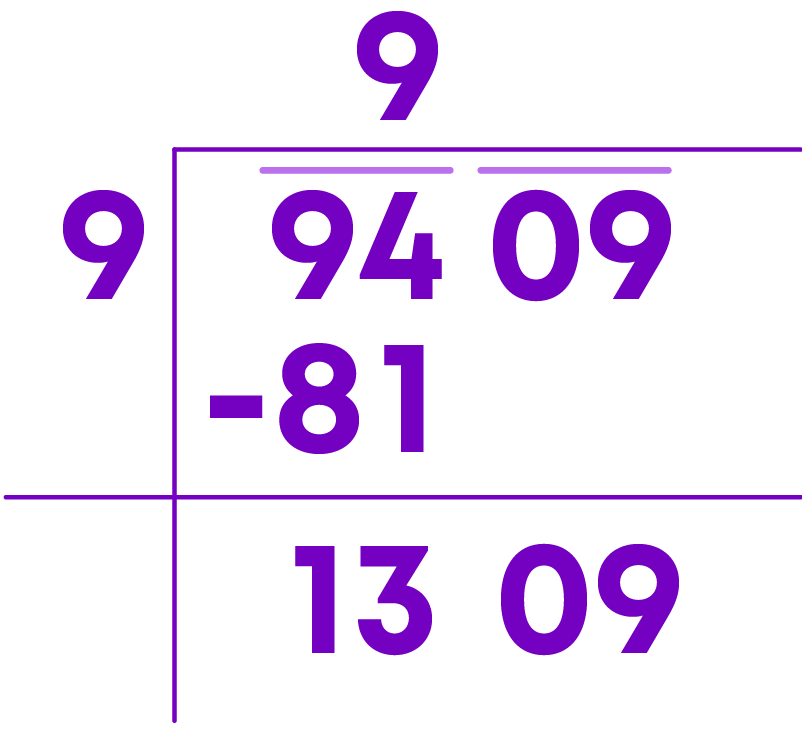Step 4: Add the divisor and quotient (9 + 9 = 18) and put a blank on the right of the number. The blank is for the next digit of the new divisor.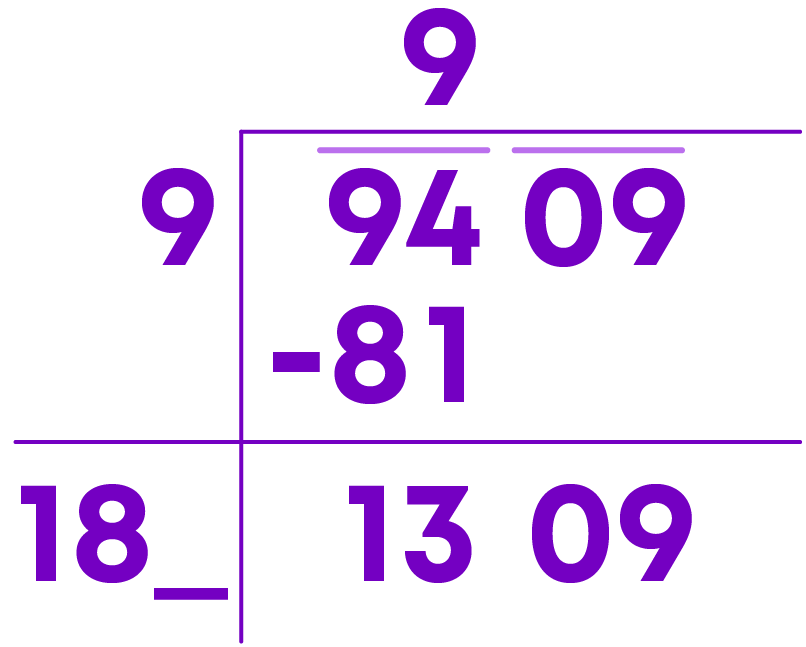Step 5: Find the largest possible digit which will fill the blank and will also be the quotients next digit. Any of the following digit can replace the blank -

(181 × 1 = 181, 182 × 2 = 364, 183 × 3 = 549, 184 × 4 = 736, 185 × 5 = 925, 186 × 6 = 1116, 187 × 7 = 1309)

Since the dividend is 1309 and 187 × 7 = 1309, the possible digit to fill in the blank is 7.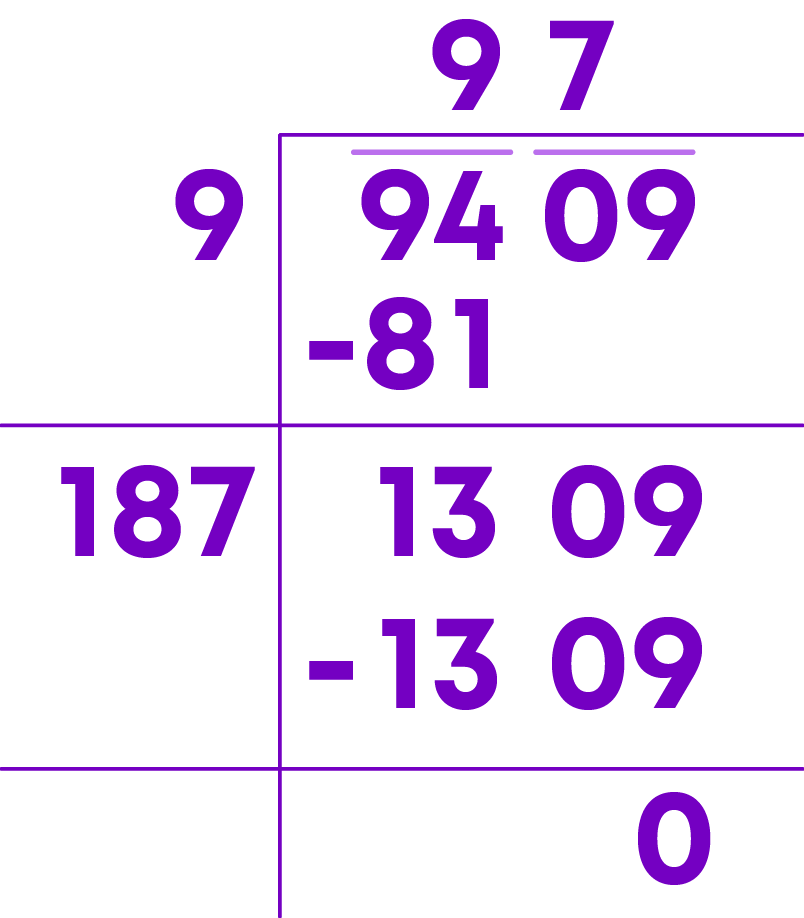We got the remainder as ‘0’ and hence the long division process is complete. The quotient of the long division method gives the square root of the number.

Hence, √9409 = 97

You already know that if a perfect square has ‘n’ digits, then its square root will have n 2 digits if n is even, and n+12 2 digits if n is odd.

As you saw in the previous example, √9409 = 97

The square root of a 4-digit number = 4 ÷ 2 = 2-digit number. Also, the square root of a 3-digit number = (3 + 1) ÷ 2 = 2-digit number.

Hence, we group the digits in pairs to match the number of digits of the square root.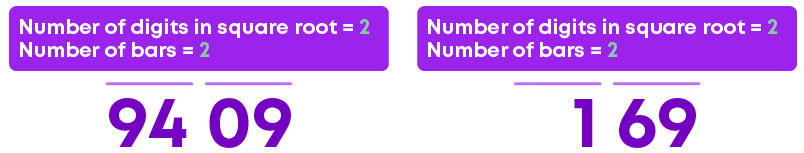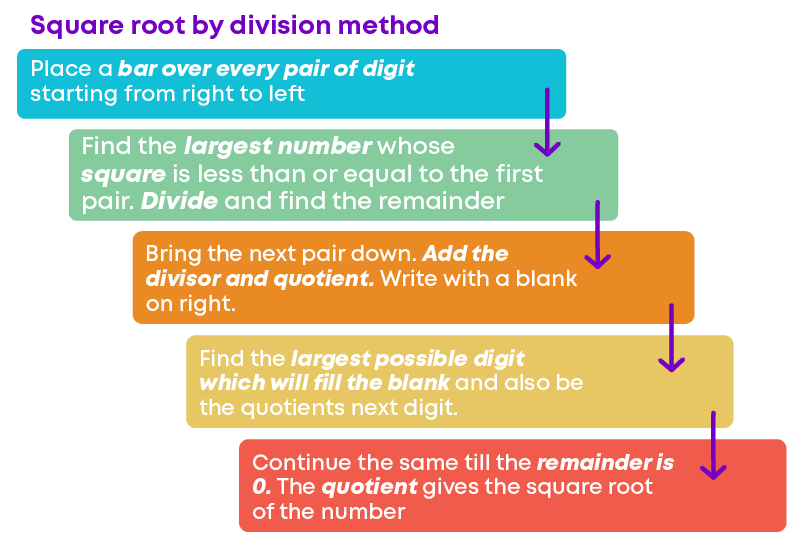How will you find the square root of a decimal number like 156.25?

Step 1: Place a bar over every pair of digits, in the integral part, starting from the right to the left.

On the decimal part of the number, place the bar over every pair of digits from the left to the right.Step 2: Start finding the square root by division method in the usual manner.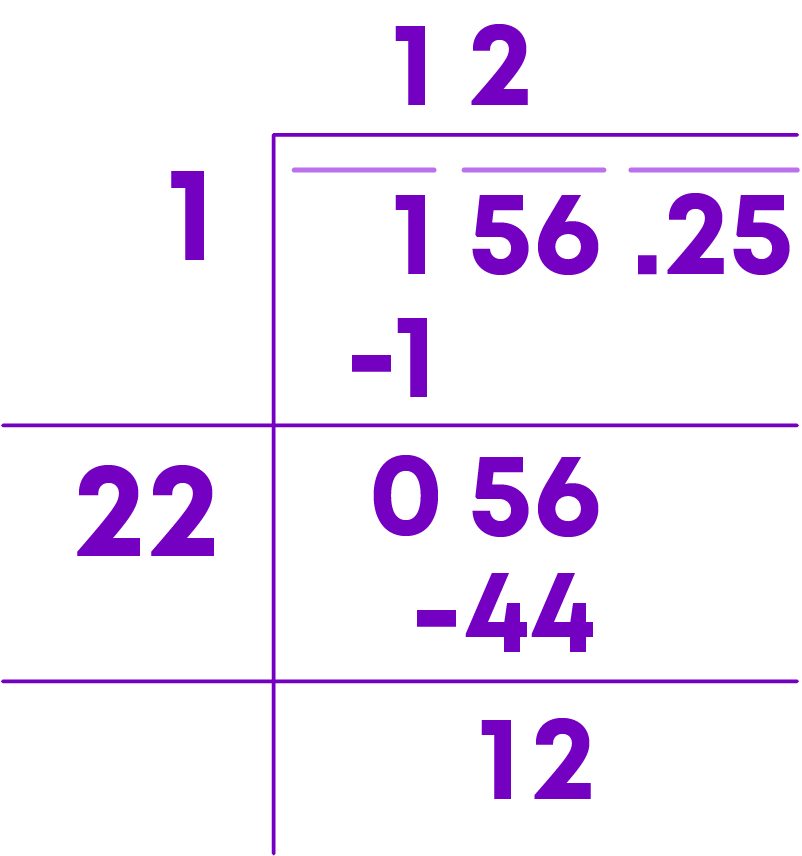Step 3: Place the decimal point in the quotient when the integral part is exhausted.

When we try to bring down the next pair of digits, we see that there is a decimal place in the dividend. Hence, we place a decimal point in the quotient. Then continue the long division method.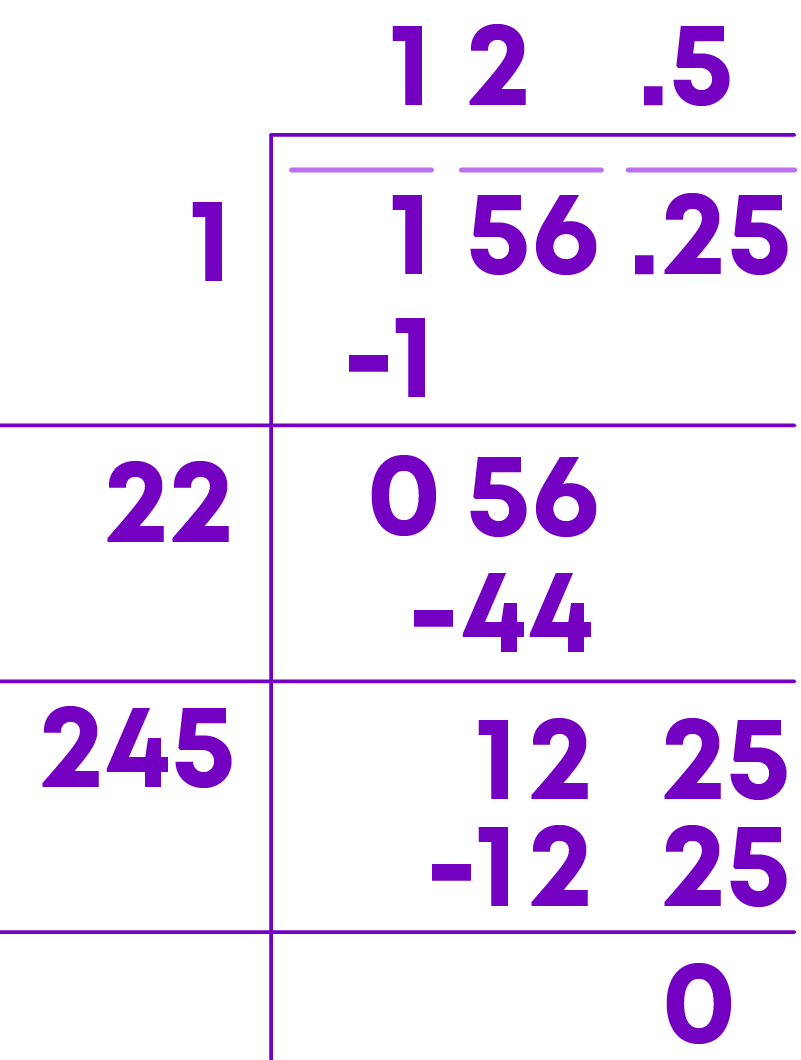We got the remainder as ‘0’ and the long division process is complete. The quotient of the long division method gives the square root of the number.

Hence, √156.25 = 12.5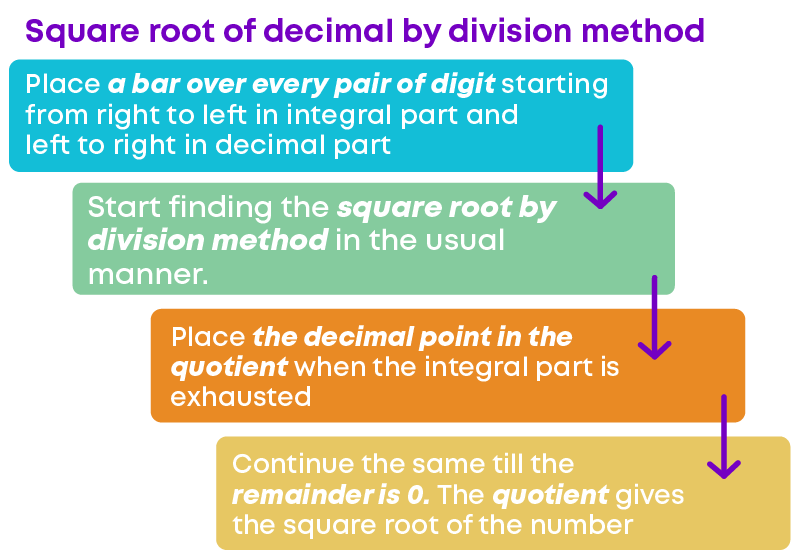There are 2 types of questions where you will use the method of prime factorisation to find the square root of numbers.

Type 1: To find the smallest number to be multiplied by the given number to get a perfect square.

The strategy is to:

• Prime factorise the given number.
• Identify the prime factors that do not occur in pairs.
• Find the product of such prime numbers.
• Multiply the smallest number obtained to the given number and then find its square root.

Type 2: To find the smallest number by which the given number is to be divided to get a perfect square.

The strategy is to:

• Prime factorise the given number.
• Identify the prime factors that do not occur in pairs.
• Find the product of such prime numbers.
• Divide the given number by this smallest number obtained. Then find its square root.

Given some numbers, we can also find the smallest perfect square number which is divisible by all the given numbers. To find the smallest perfect square number divisible by the given numbers, the strategy is to:

• Find the LCM of the given numbers.
• Prime factorise the LCM.
• Identify the prime factors that do not occur in pairs.
• Find the product of such prime numbers.
• Multiply the LCM by the product of primes in the previous step.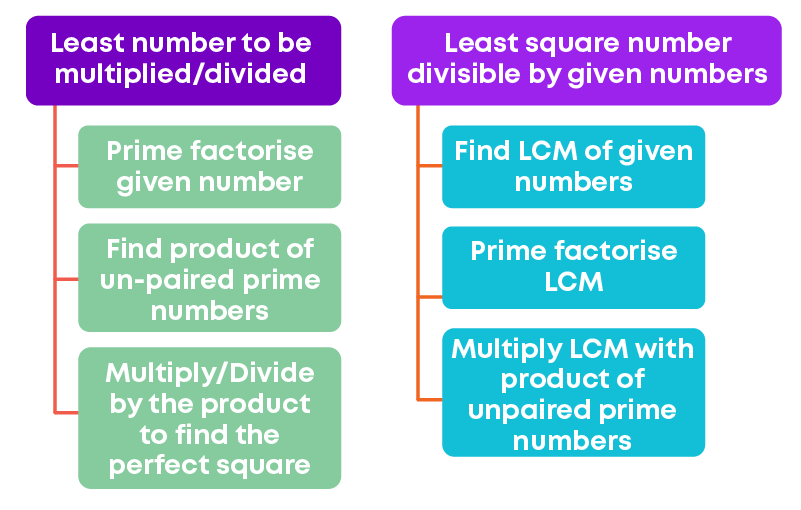There are 2 types of questions where the method of long division is used to find the square root of numbers.

Type 1: To find the smallest number to be added to the given number(N) to get a perfect square.

The strategy is to:

• Find the square root of the number N by long division.
• Identify the quotient(Q)
• The number to be added is (Q + 1)2 – N
• The square root of the square number so obtained is (Q + 1)

Type 2: To find the smallest number by which the given number(N) is to be subtracted to get a perfect square.

The strategy is to:

• Find the square root of the number N by long division.
• Identify the remainder
• The number to be subtracted is the remainder R.
• The square root of the square number so obtained is the quotient Q

We can also use long division to find the least and greatest n-digit perfect square number. There are 2 types of questions where the method of long division is used to find the least or greatest n-digit perfect square number.

Type 1: To find the smallest n-digit perfect square number

The strategy is to:

• Find the square root of the least n-digit number by long division method
• Identify the quotient(Q)
• The least-square number is (Q + 1)2
• The square root of the square number so obtained is (Q + 1)

Type 2: To find the largest n-digit perfect square number

The strategy is to:

• Find the square root of the greatest n-digit number by long division method
• Identify the remainder (R)
• Subtract the remainder from the greatest n-digit number.
• This gives the greatest n-digit square number

Remember: We use the concept of square roots in the following scenarios-

• When some arrangement is done in the form of a square and the measurement along one side must be found.
• When there are geometrical problems involving squares, rectangles, and right-angled triangles.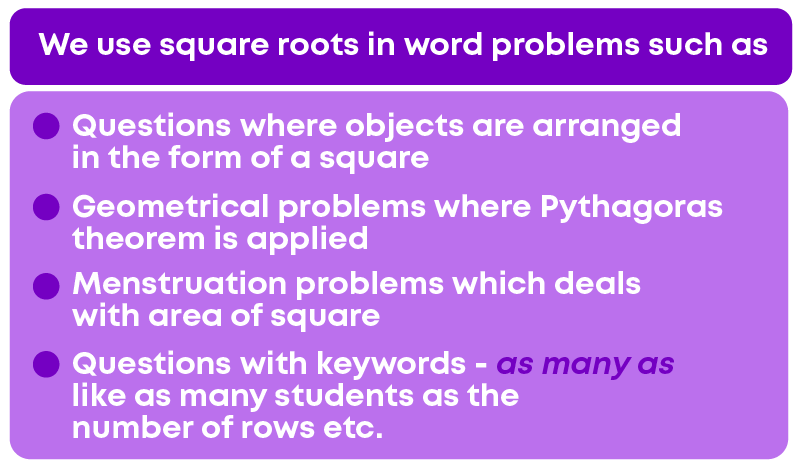#### Common Errors

The following are topics in which students make common mistakes when dealing with squares and square roots:

• 1. Pair the numbers from the right to the left
• 2. Bring down a pair
• 3. Prime factorisation or long division?

#### Pair The Numbers From The Right To The Left

To find the square root of a number by the long division method, the first step is to pair the numbers. Always pair the numbers from the right to the left.

For example, to find the square root of 10609, make groups of two digits starting from the units place as given below.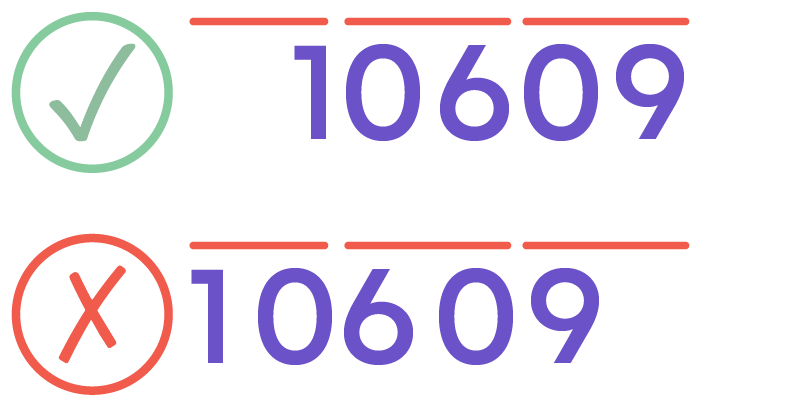#### Bring Down A Pair

To find the square root of a number by the long division method, before proceeding to the next step, we should bring down the next pair of digits. Care should be taken to bring down the whole pair and not the single digit.

For example, bring down the pair ‘09’ and not the single digit ‘0’.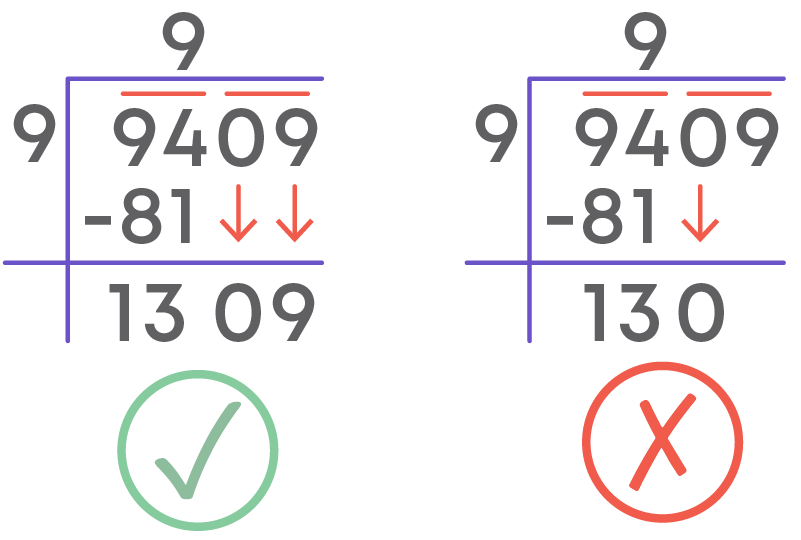#### Prime Factorisation Or Long Division?

You might have seen questions like these:

• Find the smallest number by which 1323 should be multiplied to get a perfect square.
• Find the smallest number by which 1200 should be divided to get a perfect square.
• Find the least number that must be added to 8400 to obtain a perfect square.
• Find the least number that must be subtracted from 2509 to make it a perfect square.

Are you confused as to which method is to be used to solve these types of problems?

To find the smallest number to be multiplied or divided to make a number a perfect square, use the prime factorisation method.

To find the smallest number to be added or subtracted to make a number a perfect square, use the long division method.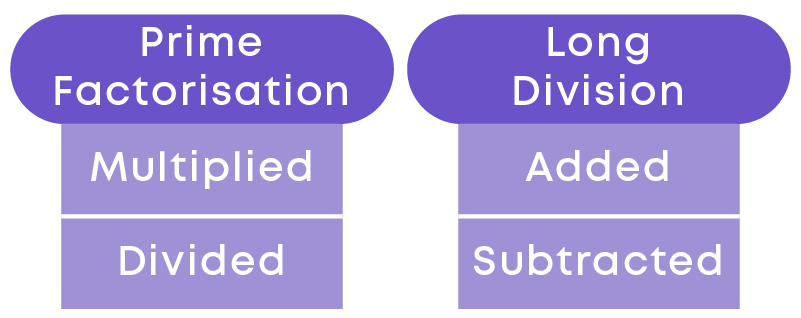#### Conclusion

Can you solve this riddle based on squares and square roots?

Observe the figure below and find the value of the question mark: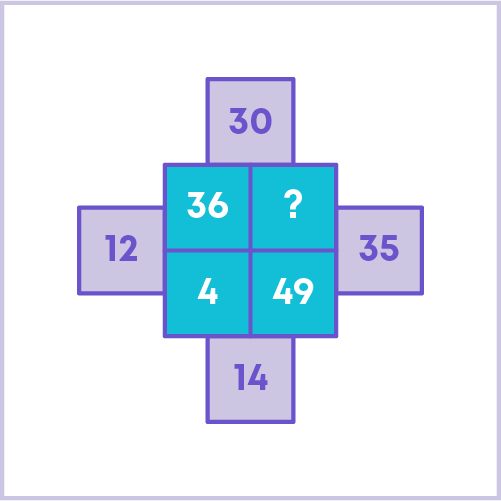#### Jyothi

Author

A math enthusiast and an educationist, Jyothi has a strong academic background and has demonstrated an impressive history of working in the education industry. Jyothi is skilled in developing and reviewing lesson plans, learning content, assessments, and video story boards and scripts.